This free HTML version of Python from the Very Beginning lives at https://pythonfromtheverybeginning.com/
(PDF or ePub $14.99, Kindle$9.99, Paperback $19.99) NOT FOR REDISTRIBUTION If you enjoy this free book, please leave a review on Amazon, or buy a paper or Kindle copy or PDF or ePub for yourself or a friend. # Python from the Very Beginning In Python from the Very Beginning John Whitington takes a no-prerequisites approach to teaching a modern general-purpose programming language. Each small, self-contained chapter introduces a new topic, building until the reader can write quite substantial programs. There are plenty of questions and, crucially, worked answers and hints. Python from the Very Beginning will appeal both to new programmers, and to experienced programmers eager to explore a new language. It is suitable both for formal use within an undergraduate or graduate curriculum, and for the interested amateur. John Whitington founded a company which sells software for document processing. He taught programming to students of Computer Science at the University of Cambridge. His other books include the textbooks “PDF Explained” (O’Reilly, 2012), “OCaml from the Very Beginning” (Coherent, 2013), and “Haskell from the Very Beginning” (Coherent, 2019) and the Popular Science book “A Machine Made this Book: Ten Sketches of Computer Science” (Coherent, 2016). C O H E R E N T P R E S S Cambridge Published in the United Kingdom by Coherent Press, Cambridge Copyright Coherent Press 2020 This publication is in copyright. Subject to statutory exception no reproduction of any part may take place without the written permission of Coherent Press. First published October 2020 A catalogue record for this book is available from the British Library by the same author PDF Explained (O’Reilly, 2012) OCaml from the Very Beginning (Coherent, 2013) More OCaml: Methods, Algorithms & Diversions (Coherent, 2014) A Machine Made this Book: Ten Sketches of Computer Science (Coherent, 2016) Haskell from the Very Beginning (Coherent, 2019) # Preface I have tried to write a book which has no prerequisites – and with which any intelligent person ought to be able to cope, whilst trying to be concise enough that a programmer experienced in another language might not be too annoyed by the pace or tone. This may well not be the last book you read on Python, but one of the joys of Python is that substantial, useful programs can be constructed quickly from a relatively small set of constructs. There is enough in this book to build such useful programs, as we see in the four extended projects. Answers and Hints are at the back of the book. ## Chapters In chapter 1 we begin our exploration of Python with a series of preliminaries, introducing ways to calculate with the whole numbers, compare them with one another, and print them out. We learn about truth values and the other types of simple data which Python supports. In chapter 2 we build little Python programs of our own, using functions to perform calculations based on changing inputs. We make decisions using conditional constructs to choose differing courses of action. In chapter 3 we learn about Python constructs which perform actions repeatedly, for a fixed number of times or until a certain condition is met. We start to build larger, more useful programs, including interactive ones which depend upon input from the keyboard. In chapter 4 we begin to build and manipulate larger pieces of data by combining things into lists, and querying and processing them. This increases considerably the scope of programs we can write. In chapter 5 we expand our work with lists to manipulate strings, splitting them into words, processing them, and putting them back together. We learn how to sort lists into order, and how to build lists from scratch using list comprehensions. In chapter 6 we learn more about printing messages and data to the screen, and use this knowledge to print nicely-formatted tables of data. We learn how to write such data to a file on the computer, instead of to the screen. In chapter 7 we learn another way of storing data – in dictionaries which allow us to build little databases, looking up data by searching for it by name. We also work with sets, which allow us to store collections of data without repetition, in the same way as mathematical sets do. In chapter 8 we deal with the thorny topic of errors: what do we do when an input is unexpected? When we find a number when we were expecting a list? When an item is not found in a dictionary? We learn how to report, handle, and recover from these errors. In chapter 9 we return to the subject of files, learning how to read from them as well as write to them, and illustrate with a word counting program. We deal with errors, such as the unexpected absence of a file. In chapter 10 we talk about real numbers, which we have avoided thus far. We show how to calculate with the trigonometric functions and how to convert between whole and real numbers by rounding. In chapter 11 we introduce the Python Standard Library, greatly expanding the pre-built components at our disposal. We learn how to look up functions in Python’s official documentation. In chapter 12 we build stand-alone programs which can be run from the command line, as if they were built in to the computer. We are now ready to begin on larger projects. ## Projects In project 1 we draw all sorts of pretty pictures by giving the computer instructions on how to draw them line by line. We make a graph plotter and a visual clock program. In project 2 we write a calorie-counting program which stores its data across several files, and which allows multiple users. We build an interface for it, with several different commands. We learn how to use a standard data format, so that spreadsheet programs can load our calorie data. In project 3 we investigate the childhood game of Noughts and Crosses, writing human and computer players, and working out some statistical properties of the game by building a structure containing all possible games. In project 4 we learn how to manipulate photographs, turning them into greyscale, blurring them, and making animations from them. ## Online resources To save typing, all the examples and exercises for this book can be found in electronic form at https://pythonfromtheverybeginning.com. The book’s errata lives there too. ## Acknowledgements The technical reviewer provided valuable corrections and suggestions, but all mistakes remain the author’s. The image of strategy for Noughts and Crosses is from “Flexible Strategy Use in Young Children’s Tic‐Tac‐Toe” by Kevin Crowley and Robert S. Siegler, and is reproduced courtesy of Elsevier. # Getting Ready This book is about teaching the computer to do new things by writing computer programs. Just as there are different languages for humans to speak to one another, there are different programming languages for humans to speak to computers. We are going to be using a programming language called Python. A Python system might already be on your computer, or you may have to find it on the internet and install it yourself. You will know that you have it working when you see something like this: Python 3.8.2 (default, Feb 24 2020, 18:27:02) [Clang 10.0.1 (clang-1001.0.46.4)] on darwin Type "help", "copyright", "credits" or "license" for more information. >>>  Make sure the Python version number in the first line, here Python 3.8.2, is at least 3. You might need to type python3 instead of python to achieve this. Python is waiting for us to type something. Try typing 1 + 2 followed by the Enter key. You should see this: Python >>> 1 + 2 3 >>>  (We have abbreviated Python’s welcome message). Python tells us the result of the calculation. You may use the left and right arrow keys on the keyboard to correct mistakes and the up and down arrow keys to look through a history of previous inputs. You can also use your computer’s usual copy and paste functions, instead of typing directly into Python, if you like. To abandon typing, and ask Python to forget what you have already typed, enter Ctrl-C (hold down the Ctrl key and tap the c key). This will allow you to start again. To leave Python altogether, give the exit() command, again followed by the Enter key: Python >>> exit() You should find yourself back where you were before. We are ready to begin. # 1. Starting Off We will cover a fair amount of material in this chapter and its questions, since we will need a solid base on which to build. You should read this with a computer running Python in front of you. ### Expressions and statements A computer program written in Python is built from statements and expressions. Each statement performs some action. For example, the built-in print statement writes to the screen: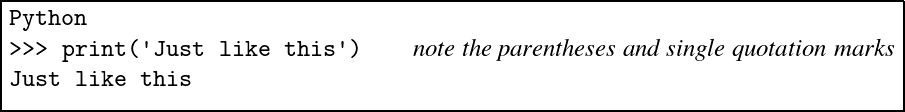Each expression performs some calculation, yielding a value. For example, we can calculate the result of a simple mathematical expression using whole numbers (or integers): Python >>> 1 + 2 * 3 7 When Python has calculated the result of this expression, it prints it to the screen, even though we have not used print. All of our programs will be built from such statements and expressions. The single quotation marks in our print statement indicate that what we are printing is a string – a sequence of letters or other symbols. If the string is to contain a single quotation mark, we must use the double quotation mark key instead: Python >>> print('Can't use single quotation marks here!') File "<stdin>", line 1 print('Can't use single quotation marks here!') ^ SyntaxError: invalid syntax >>> print("Can't use single quotation marks here!") Can't use single quotation marks here! Note that this is a different key on the keyboard – we are not typing two single quotation marks – it is " not ’ ’. We can print numbers too, of course: Python >>> print(12) 12 Note that 12 and ’12’ are different things: one is the whole number (or integer) 12, and one is the string consisting of the two symbols 1 and 2. Notice also the difference between an expression which is just a string, and the statement which is the act of printing a string: Python >>> 'Just like this' 'Just like this' >>> print('Just like this') Just like this ### Numbers We have seen how to do mathematical calculations with our numbers, of course: Python >>> 1 + 2 * 3 7 Even quite large calculations: Python >>> 1000000000 + 2000000000 * 3000000000 6000000001000000000 Using the _ underscore key to split up the numbers is optional, but helps with readability: Python >>> 1_000_000_000 + 2_000_000_000 * 3_000_000_000 6000000001000000000 Python reduces the mathematical expression 1 + 2 * 3 to the value 7 and then prints it to the screen. This expression contains the operators + and * and their operands 1, 2, and 3. How does Python know how to calculate 1 + 2 * 3? Following known rules, just like we would. We know that the multiplication here should be done before the addition, and so does Python. So the calculation goes like this: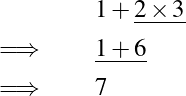The piece being processed at each stage is underlined. We say that the multiplication operator has higher precedence than the addition operator. Here are some of Python’s operators for arithmetic: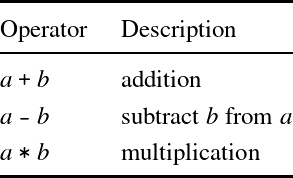In addition to our rule about * being performed before + and -, we also need a rule to say what is meant by 9 - 4 + 1. Is it (9 - 4) + 1 which is 6, or 9 - (4 + 1) which is 4? As with normal arithmetic, it is the former in Python: Python >>> 9 - 4 + 1 6 This is known as the associativity of the operators. ### Truth and falsity Of course, there are many more things than just numbers. Sometimes, instead of numbers, we would like to talk about truth: either something is true or it is not. For this we use boolean values, named after the English mathematician George Boole (1815–1864) who pioneered their use. There are just two booleans: True False How can we use these? One way is to use one of the comparison operators, which are used for comparing values to one another: Python >>> 99 > 100 False >>> 4 + 3 + 2 + 1 == 10 True It is most important not to confuse == with = as the single = symbol means something else in Python. Here are the comparison operators: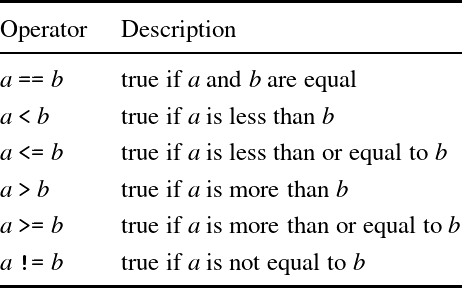There are two operators for combining boolean values (for instance, those resulting from using the comparison operators). The expression a and b evaluates to True only if expressions a and b both evaluate to True. The expression a or b evaluates to True if a evaluates to True or b evaluates to True, or both do. Here are some examples of these operators in use: Python >>> 1 == 1 and 10 > 9 True >>> 1 == 1 or 9 > 10 True In each case, the expression a will be tested first – the second may not need to be tested at all. The and operator is performed before or, so a and b or c is the same as (a and b) or c. The expression not a gives True if a is False and vice versa: Python >>> not 1 == 1 False >>> 1 == 2 or not 9 > 10 True The comparison operators have a higher precedence than the so-called logical operators: so, for example, writing not 1 == 1 is the same as writing not (1 == 1) rather than (not 1) == 1. ### The types of things In this chapter we have seen three types of data: strings, integers and booleans. We can ask Python to tell us the type of a value or expression: Python >>> type('Hello!') <class 'str'> >>> type(25) <class 'int'> >>> type(1 + 2 * 3) <class 'int'> >>> type(False) <class 'bool'> Here, ’str’ indicates strings, ’bool’ booleans, and ’int’ integers. ## Common problems When Python does not recognise what we type in as a valid program, an error message is shown instead of an answer. You will come across this many times when experimenting with your first Python programs, and part of learning to program is learning to recognise and fix these mistakes. For example, if we miss the quotation mark from the end of a string, we see this: Python >>> print('A string without a proper end) File "<stdin>", line 1 print('A string without a proper end) ^ SyntaxError: EOL while scanning string literal Such error messages are not always easy to understand. What is EOL? What is a literal? What is <stdin>? Nevertheless, you will become used to such messages, and how to fix your programs. In the next example, we try to compare a number to a string: Python >>> 1 < '2' Traceback (most recent call last): File "<stdin>", line 1, in <module> TypeError: '<' not supported between instances of 'int' and 'str' In this case the error message is a little easier to understand. Another common situation is missing out a closing parenthesis. In this case, Python does not know we have finished typing, even when we press Enter. Python >>> 2 * (3 + 4 + 5 ... ... ... ...  To get out of this situation, we can type Ctrl-C, to let Python know we wish to discard the statement and try again: Python >>> 2 * (3 + 4 + 5 ... ... ... ... KeyboardInterrupt Or, if possible, we can finish the expression properly: Python >>> 2 * (3 + 4 + 5 ... ... ... ...) 24 ## Summary We have learned how to interact with Python by typing statements and reading the answers. We have learned about three types of data: strings, whole numbers, and booleans. We have seen how to perform arithmetic on numbers, and how to test things for equality with one another, using operators and operands. We have learned about boolean operators too. Finally, we have learned how to ask Python to tell us the type of something. In the next chapter, we will move on to more substantial programs. Meanwhile, there are some questions to try. Answers and hints are at the back of the book. ## Questions 1. What sorts of thing do the following expressions represent and to what do they evaluate, and why? See if you can work them out without the computer to begin with. 17 1 + 2 * 3 + 4 400 > 200 1 != 1 True or False True and False ’%’ 2. A programmer writes 1+2 * 3+4. What does this evaluate to? What advice would you give them? 3. Python has a modulus or remainder operator, which finds the remainder of dividing one number by another. It is written %. Consider the evaluations of the expressions 1 + 2 % 3, (1 + 2) % 3, and 1 + (2 % 3). What can you conclude about the + and % operators? 4. What is the effect of the comparison operators like < and > on strings? For example, to what does ’bacon’ < ’eggs’ evaluate? What about ’Bacon’ < ’bacon’? What is the effect of the comparison operators on the booleans True and False? 5. What (if anything) do the following statements print on the screen? Can you work out or guess what they will do before typing them in? 1 + 2 ’one’ + ’two’ 1 + ’two’ 3 * ’1’ ’1’ * 3 print(’1’ * 3) True + 1 print(f’One and two is {1 + 2} and that is all.’) (The last of these uses Python in a way we have not yet mentioned.) # 2. Names and Functions So far we have built only tiny toy programs. To build bigger ones, we need to be able to name things so as to refer to them later. We also need to write expressions whose result depends upon one or more other things. ### Names So far, if we wished to use a sub-expression twice or more in a single expression, we had to type it multiple times: Python >>> 200 * 200 * 200 8000000 Instead, we can define our own name to stand for the result of evaluating an expression, and then use the name as we please: Python >>> x = 200 >>> x * x * x 8000000 We can update the value associated with the name and try the calculation again: Python >>> x = 200 >>> x * x * x 8000000 >>> x = 5 + 5 >>> x * x * x 1000 Because of this ability to vary the value associated with the name, things like x are called variables. We can use any name we like for a variable, so long as it does not clash with any of Python’s built in keywords: and as assert async await break class continue def del elif else except finally for from global if import in is lambda nonlocal not or pass raise return try while with yield In Python, we use lower case letters or words for variable names. For example x, weight, or total. If we wish to use multiple words, we separate them with underscores. For example first_string or total_of_subtotals. ### Functions We can make a function, whose value depends upon some input. We call this input an argument – we will be using the word “input” later in the book to mean something different: Python >>> def cube(x): return x * x * x ... >>> cube(10) 1000 >>> answer = cube(20) >>> answer 8000 Note that we had to press the Enter key twice when defining the function: we shall discover why momentarily. What are the parts to this definition of the function cube? We write def, followed by the function name, its argument in parentheses, and a colon. Then, we calculate x * x * x and use return to return the value to us. We need the word return because not all functions return something. For example, this function prints a string given to it twice to the screen, but does not return a value: Python >>> def print_twice(x): ... print(x) ... print(x) ... >>> print_twice('Ha') Ha Ha >>> print_twice(1) 1 1 Notice this function spans multiple lines. It can operate on both strings and numbers. Now you can see why we needed to press Enter twice when defining the cube and print_twice functions – so that Python knows when we have finished entering a multi-line function. ### Indentation Each of the print(x) lines in print_twice is indented (moved to the right by insertion of four spaces). This helps us to show the structure of the program more clearly, and in fact is a requirement – Python will complain if we do not do it: Python >>> def print_twice(x): ... print(x) File "<stdin>", line 2 print(x) ^ IndentationError: expected an indented block You will come across this error frequently as you learn to indent your Python programs correctly. ### Functions with choices We can use the keywords if and else to build a function which makes a choice based on some test. For example, here is a function which determines if an integer is negative: Python >>> def neg(x): ... if x < 0: ... return True ... else: ... return False We can test it like this: Python >>> neg(1) False >>> neg(-1) True Notice the indentation of each part of this function, after every line which ends with a colon – again, it is required. We can write it using fewer lines, if it will fit: Python >>> def neg(x): ... if x < 0: return True ... else: return False Of course, our function is equivalent to just writing Python >>> def neg(x): ... return x < 0 because x < 0 will evaluate to the appropriate boolean value on its own – True if x < 0 and False otherwise. Here is another function, this time to determine if a given string is a vowel or not: Python >>> def is_vowel(s): ... return s == 'a' or s == 'e' or s == 'i' or s == 'o' or s == 'u' >>> is_vowel('x') False >>> is_vowel('u') True If we need to test for more than one condition we can use the elif keyword (short for “else if”): Python >>> def sign(x): >>> if x < 0: return -1 >>> elif x == 0: return 0 >>> else: return 1 This function returns the sign of a number, irrespective of its magnitude. Of course, with extra indenting, this could be written without elif. Can you see how? ### Multiple arguments There can be more than one argument to a function. For example, here is a function which checks if two numbers add up to ten: Python >>> def add_to_ten(a, b): ... return a + b == 10 >>> add_to_ten(6, 4) True >>> add_to_ten(6, 5) False The result is a boolean. We use the function in the same way as before, but writing two numbers this time, one for each argument the function expects. Finally, let us use the + operator in a different way, to concatenate strings: Python >>> def welcome(first, last): ... print('Welcome, ' + first + ' ' + last + '! Enjoy your stay.') >>> welcome('Richard', 'Smith') Welcome, Richard Smith! Enjoy your stay. ### Going round again A recursive function is one which uses itself in its own definition. Consider calculating the factorial of a given number – for example the factorial of 4 (written 4! in mathematics) is 4 × 3 × 2 × 1. Here is a recursive function to calculate the factorial of a positive number. Python >>> def factorial(a): ... if a == 1: ... return 1 ... else: ... return a * factorial(a - 1) For example: Python >>> factorial(4) 24 >>> factorial(100) 933262154439441526816992388562667004 907159682643816214685929638952175999 932299156089414639761565182862536979 208272237582511852109168640000000000 00000000000000 How does the evaluation of factorial(4) proceed?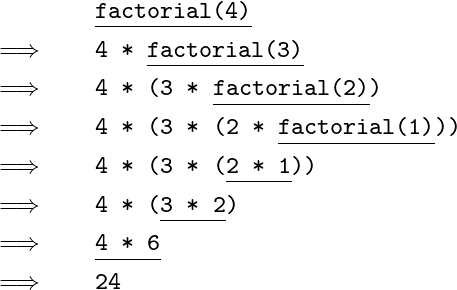For the first three steps, the else part of the conditional expression is chosen, because the argument a is greater than one. When the argument is equal to one, we do not use factorial again, but just evaluate to 1. The expression built up of all the multiplications is then evaluated until a value is reached: this is the result of the whole evaluation. It is sometimes possible for a recursive function never to finish – what if we try to evaluate factorial(-1)?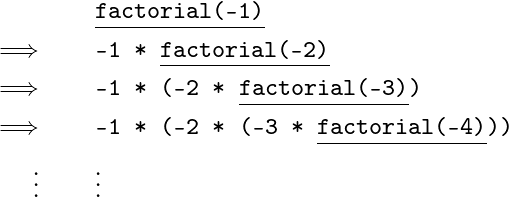The expression keeps expanding, and the recursion keeps going. Helpfully, Python tells us what is going on: Python >>> factorial(-1) Traceback (most recent call last): File "<stdin>", line 1, in <module> File "<stdin>", line 5, in factorial File "<stdin>", line 5, in factorial File "<stdin>", line 5, in factorial [Previous line repeated 995 more times] File "<stdin>", line 2, in factorial RecursionError: maximum recursion depth exceeded in comparison We do not use recursive functions often in Python, preferring the methods of repeated action described in the next chapter. But it can be interesting to think about how they work, and some of the questions at the end of the chapter invite you to do just that. Almost every program we write will involve functions such as those shown in this chapter, and many larger ones too – using functions to split up a program into small, easily understandable chunks is the basis of good programming. ## Common problems Now that we are writing slightly larger programs which might span multiple lines, new types of mistake are available to us. A common mistake is to forget the colon at the end of a line. For example, here we forget it after an if: Python >>> def neg(x): ... if x < 0 File "<stdin>", line 2 if x < 0 ^ SyntaxError: invalid syntax Syntax is a word for the arrangement of symbols and words to make a valid program. If we forget the proper indentation, Python complains too: Python >>> def neg(x): ... if x < 0: ... return True File "<stdin>", line 3 return True ^ IndentationError: expected an indented block We must also remember to avoid using one of Python’s keywords as a variable or function name, even in an otherwise valid program: Python >>> def class(x): return '30 pupils' File "<stdin>", line 1 def class(x): return '30 pupils' ^ SyntaxError: invalid syntax Another common mistake is to omit the return in a function: Python >>> def double(x): x * 2 ... >>> double(5) >>> In this case, Python accepts the function, and we only discover our mistake when we try to use it. ## Summary We have learned how to give names to our values so as to use and reuse them in different contexts, and to update the values associated with such names. We have written functions whose result depends upon one or more arguments, including multi-line functions. We have seen how to choose a course of action based upon testing the value associated with a name. Finally, we have experimented with recursive functions to perform repeated processing of one or more arguments. We have explained, though, that recursion is not ordinarily used in Python. In the next chapter, we will introduce the standard Python mechanisms for repeated actions or calculations. ## Questions Questions 5–8 are optional – we do not often use recursive functions in Python. 1. Write a function which multiplies a given number by ten. 2. Write a function which returns True if both of its arguments are non-zero, and False otherwise. 3. Write a function volume which, given the width, height, and depth of a box, calculates its volume. Write another function volume_ten_deep which fixes the depth at 10. It should be implemented by using your volume function. 4. Write a function is_consonant which, given a lower-case letter in the range ’a’’z’, determines if it is a consonant. 5. Can you suggest a way of preventing the non-termination of the factorial function in the case of a zero or negative argument? 6. Write a recursive function sum_nums which, given a number n, calculates the sum 1 + 2 + 3 + … + n. 7. Write a recursive function power(x, n) which raises x to the power n. 8. Write a recursive function to list the factors of a number. For example, factors(12) should print: 1 2 3 4 6 12 # Using Scripts From now on, instead of showing the actual Python session… Python >>> def factorial(n): ... if n == 1: ... return n ... else: ... return n * factorial(n - 1) …we will usually just show the program in a box: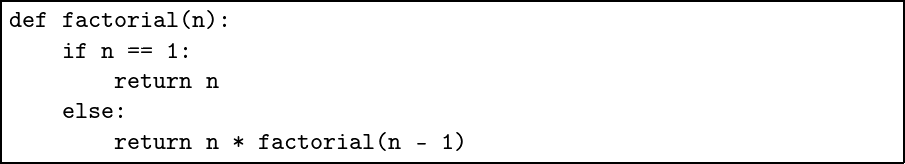In fact, this is just how Python programs are normally written, in a text file with the .py extension, rather than typed directly into Python. We can use the from … import … construct to access the program from Python. Assuming we have a file script.py which looks like the contents of the box above, we can write: Python >>> from script import factorial >>> factorial(4) 24 We can use from … import * to import all definitions from a script. When we have made a change to the file script.py in our text editor (and saved the file), Python must be restarted and the script imported anew. You will notice that, after running the import statement, the directory __pycache__ has appeared alongside script.py. This is for Python’s internal use, and you may discard it if you like. # 3. Again and Again In the previous chapter we used recursion to perform a calculation a variable number of times, and noted its limitations in Python. In this chapter, we learn the ordinary Python way of handling such situations. ### A fixed number of times The for … in range(a, b) structure can be used to do something a number of times. For example, to print each of the numbers in the range in turn: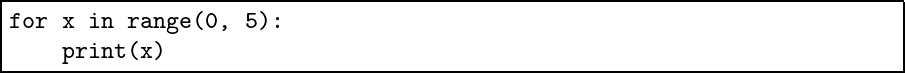The expressions inside the for construct (or loop, as we call it) will be run once for each number in the range. Here is what we see on the screen: 0 1 2 3 4 We can see that the two arguments to the range function specify where to start, at 0, and where to stop, before 5. And so, the numbers 0 to 4 inclusive are printed. This behaviour is useful when programming, but unintuitive to humans. Let us write a function to print the numbers 1…n as a human might expect: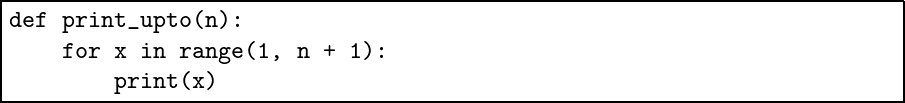So now, print_upto(5) will print this: 1 2 3 4 5 What if we want the numbers to be printed all on the same line? The Python print function moves to the next line by default. We can suppress this behaviour by supplying an alternative end to the line (print usually ends the line with what is called a newline character):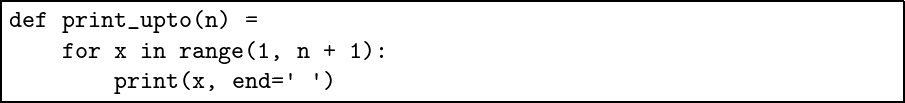Now the same statement print_upto(5) will print the numbers all on one line, with spaces in between: 1 2 3 4 5 We have used a second argument to the built-in print function – it is a named argument, with the name end. Such names help us remember which argument is which. ### One inside another We can, of course, put one for loop inside another. Let us write a function to print a times table of any size: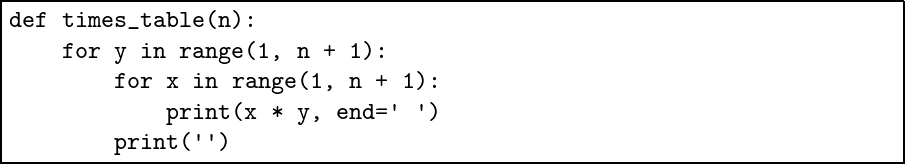We have used print with the empty string as its argument to move to a new line. Notice how the indentation helps to show the structure of the nested for structures. Here is the output of our new function for table size 5: 1 2 3 4 5 2 4 6 8 10 3 6 9 12 15 4 8 12 16 20 5 10 15 20 25 The columns are not lined up nicely, because some numbers need one digit to print and some need two. We can use the special letter \t, called a tab, to line the letters up (the \ is called the escape character, and gives the letter following it special significance.)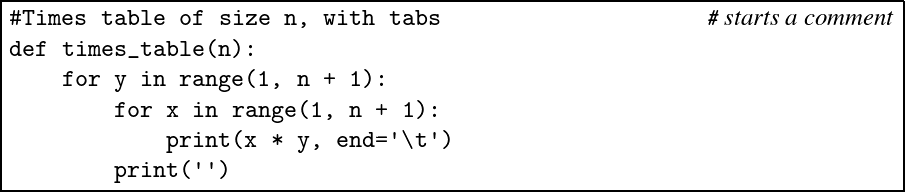Notice we have added a comment (beginning with #) to remind us that this is a different version of times_table. You can put as many comments as you like in your programs. Here is the output: 1 2 3 4 5 2 4 6 8 10 3 6 9 12 15 4 8 12 16 20 5 10 15 20 25 Tabs are a remnant of mechanical typewriter technology, where little metal stops could be placed in certain positions to line up columns at the touch of a button. We can do better by calculating the maximum width of any column, then printing enough spaces after each number: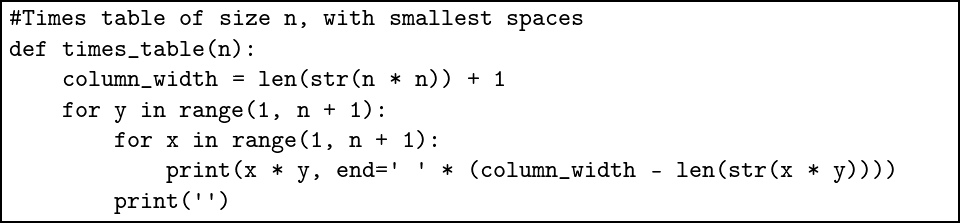The built-in function str converts a number to a string, and the built-in function len calculates the length of a string. We also use the * operator to build the string of many spaces from one space. For example, ’ ’ * 5 is ’ ’. Here is the output: 1 2 3 4 5 2 4 6 8 10 3 6 9 12 15 4 8 12 16 20 5 10 15 20 25  Much better. ### Ranging over strings We can also use for to loop or iterate over things other than ranges of numbers. For example, if we use for… in with a string, each letter of the string will be selected in turn: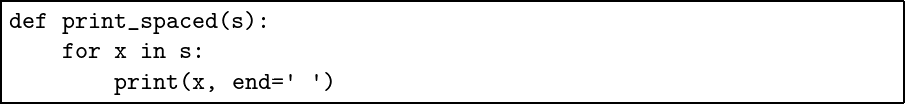The output of print_spaced(’CHARLES’) will be C H A R L E S . In one of the questions you will be asked to find a way to remove that errant last space. ### An unknown number of times What if we do not know how many times to repeat an action until we begin? We can use the while construct. For example, let us ask the user for a password before proceeding: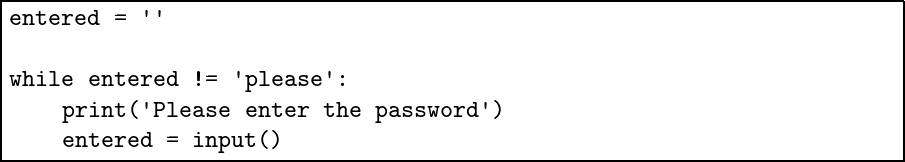The built-in input function, which has no arguments, allows the user to type in a line of text, returning when the Enter key is pressed. Here is a possible interaction: Please enter the password no Please enter the password password Please enter the password please We cannot know how many times we might need to prompt the user for input, and so we could not have done this with a for loop. We can build our while loop into a function: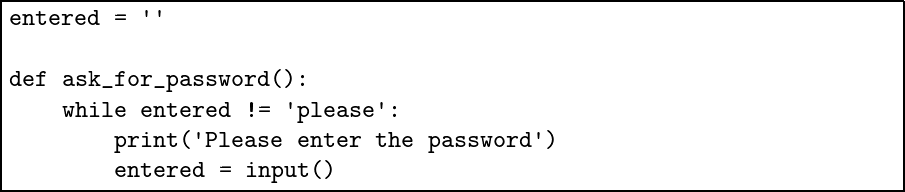Notice that our function also has no arguments, just like input, and returns nothing. However, it does not work: Python >>> ask_for_password() Traceback (most recent call last): File "<stdin>", line 1, in <module> File "<stdin>", line 2, in ask_for_password UnboundLocalError: local variable 'entered' referenced before assignment ### Local and global names To write this function correctly, we must bring the definition of the entered variable inside the function definition – it will be a new, empty, entered each time the function is run: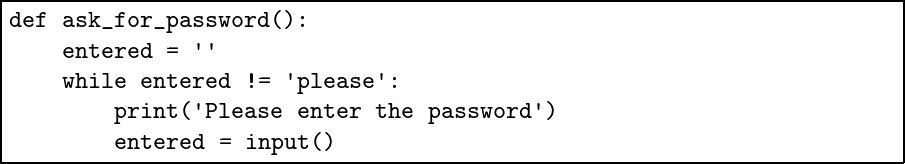This is called a local variable. In our first example, entered was a global variable. If we really wanted to use a global variable, we would write: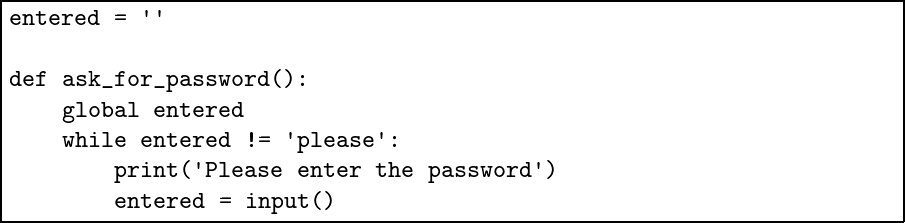There is a flaw in this program, however. If we run this version of the ask_for_password function twice then, on the second run, the variable entered will already have the correct password in it. So it is right that Python warns us of the dangers of global variables by requiring them to be explicitly declared. We will not use global in this book again. ## Common problems It is important when building for loops to remember range, or we may be in for a nasty surprise: Python >>> for x in (0, 5): ... print(x) ... 0 5 As we have already mentioned, it is important to remember that ranges begin at the first number given and stop before the second number given: Python >>> for x in range(1, 10): ... print(x) ... 1 2 3 4 5 6 7 8 9 These are called half-open intervals. They are unintuitive to the beginner, but to the experienced programmer, they are more convenient, making sure that important properties hold. For example, that len(range(a, b)) == a - b. When you begin to write programs with input, there is always the chance of problems with unexpected inputs. For example, expecting a number and using the built-in int function, which converts a string into a number: Python >>> a = input() bob >>> int(a) Traceback (most recent call last): File "<stdin>", line 1, in <module> ValueError: invalid literal for int() with base 10: 'bob' Later in the book, we will see how to deal cleanly with such situations. ## Summary We have learned about two methods for repeating statements: for loops for a known number of times, and while loops when we do not know the number of times in advance. We have, along the way, converted from strings and numbers and back again with str and int, learned how to customize the print function, built bigger strings from smaller ones, and calculated the length of a string. We have started to build interactive programs, accepting input from the user. We now have the tools to build a much wider and more interesting class of programs. ## Questions 1. The range construct can be given an extra, third, argument, the step. For example range(0, 10, 2) would iterate over 0, 2, 4, 6, and 8. Use this argument to write a function print_down_from which is the same as our print_upto function but prints the numbers in reverse order. 2. Our times table function, even in its final version, can put in too much space. This happens when only the last column contains the longest numbers, for example: Python >>> times_table(10) 1 2 3 4 5 6 7 8 9 10 2 4 6 8 10 12 14 16 18 20 3 6 9 12 15 18 21 24 27 30 4 8 12 16 20 24 28 32 36 40 5 10 15 20 25 30 35 40 45 50 6 12 18 24 30 36 42 48 54 60 7 14 21 28 35 42 49 56 63 70 8 16 24 32 40 48 56 64 72 80 9 18 27 36 45 54 63 72 81 90 10 20 30 40 50 60 70 80 90 100  Here only the final column has a number with three digits. Modify the function to correct this shortcoming. 3. Write a function count_spaces to count the number of spaces in a string. 4. Fix our print_spaced function to remove the errant final space. Hint: remember that the built-in function len can be used to find the length of a string. 5. Write a function which prints a sentence for the user to copy. Have the user type it in, and press Enter. Check if it is correct and print an appropriate message. If it is incorrect, keep going until it is correct. 6. Simplify our password example by supplying the prompt text directly as an argument to the input function. You will need to add the special string ’\n’, called the newline character, to the end to move to the next line. Simplify it further by finding a way to remove the entered variable. You will need to use the pass keyword, which does nothing. 7. Use the input function to write an interactive guessing game. For example, we might see: Python >>>guessing_game() Guess a number between 1 and 100 50 Lower! 15 Higher! 40 Lower! 35 Higher 37 Correct! You took 5 guesses. You will need the built-in function int which converts a string to an integer. An arbitrary number between 1 and 100 may be obtained in the following way: Python >>> import random >>> random.randint(1, 100) 44 (Note that we could also use from random import randint here and write randint instead of random.randint.) 8. Write a function to print a message in Morse code. Here is the table of codes: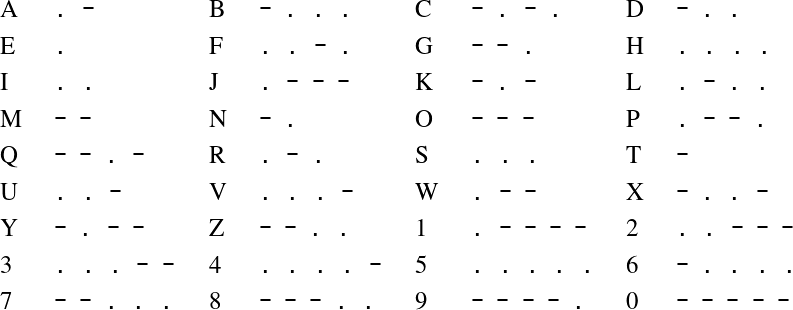There should be three spaces between letters, and seven spaces between words. # 4. Making Lists We have seen simple Python values such as numbers and strings and booleans, but we have not yet seen how to combine them into bigger structures. We do so now. ### Introducing lists A list in Python is an ordered sequence of elements. Here is the list containing the words representing the first few numbers: ['zero', 'one', 'two', 'three', 'four', 'five'] Equally, we could put numbers or booleans in our list, or nothing – the empty list is written []. Here are the first few prime numbers: [2, 3, 5, 7, 11, 13] We can find the length of the list with len, just as we used it to find the length of a string: Python >>> len([2, 3, 5, 7, 11, 13]) 6 It is possible to mix up elements of different types: [1, 'one', False] We will not be doing that in this book, however. ### Accessing elements We can fetch a single element from the list (the first element is number 0): Python >>> l = ['zero', 'one', 'two', 'three', 'four', 'five'] >>> l 'zero' >>> l 'five' >>> l Traceback (most recent call last): File "<stdin>", line 1, in <module> IndexError: list index out of range Notice the error when we go out of range. It is uncommon to write large computer programs correctly the first time, and we often have to track down and correct such errors. ### Iterating over lists We can iterate over the elements of a list with a for loop, just like we iterated of a range of numbers with range: Python >>> for x in l: ... print(x + ' has ' + str(len(x)) + ' letters.') ... zero has 4 letters. one has 3 letters. two has 3 letters. three has 5 letters. four has 4 letters. five has 4 letters. There is a connection between this mechanism and the range function we used with for loops earlier. We can use the list function to build lists from ranges: Python >>> list(range(1, 10)) [1, 2, 3, 4, 5, 6, 7, 8, 9] >>> list(range(1, 10, 3)) [1, 4, 7] In fact, we could write a for loop by making a list from a range: Python >>> for x in list(range(1, 5)): ... print(x) ... 1 2 3 4 This has the same effect as simply writing range(1, 5), but needlessly constructs the list of numbers. When we use range on its own no such intermediate list need be created. Sometimes we need both the index in the list and the item at that index. By using enumerate, and giving two names – one for the index and one for the value – we can do this easily: Python >>> for i, elt in enumerate([1, 2, 4, 8, 16]): ... print('2 to the power ' + str(i) + ' is ' + str(elt)) ... 2 to the power 0 is 1 2 to the power 1 is 2 2 to the power 2 is 4 2 to the power 3 is 8 2 to the power 4 is 16 ### List slices We can pick parts of the list out using what is called a slice. A slice is defined using start and stop positions: Python >>> l = ['zero', 'one', 'two', 'three', 'four', 'five'] >>> l[1:4] ['one', 'two', 'three'] >>> l[1:6] ['one', 'two', 'three', 'four', 'five'] >>> l[0:6] ['zero', 'one', 'two', 'three', 'four', 'five'] Notice that the stop value defines the position to stop before, just like with a range. We may omit the start or stop value. This will then be taken to stretch to the omitted end of the list: Python >>> l = ['zero', 'one', 'two', 'three', 'four', 'five'] >>> l[:4] ['zero', 'one', 'two', 'three'] >>> l[1:] ['one', 'two', 'three', 'four', 'five'] Even when the slice contains only one value, it is a list of one element, not just the element: Python >>> l = ['zero', 'one', 'two', 'three', 'four', 'five'] >>> l[4:5] ['four'] If a slice contains no values, it is the empty list []: Python >>> l = ['zero', 'one', 'two', 'three', 'four', 'five'] >>> l[4:4] [] A negative number in a slice counts from the end of the list instead: Python >>> l = ['zero', 'one', 'two', 'three', 'four', 'five'] >>> l[-3:-1] ['three', 'four'] ### Adding to a list We can add an item to the end of a list: Python >>> l = ['zero', 'one', 'two', 'three', 'four', 'five'] >>> l.append('six') >>> l ['zero', 'one', 'two', 'three', 'four', 'five', 'six'] Functions like append which are accessed by putting a dot after the value itself, are called methods. Notice that the list l is modified, rather than a new list being returned. We can concatenate lists using the same + operator used for concatenating lists and strings. Python >>> l1 = [1, 2, 3] >>> l2 = [4, 5, 6] >>> l1 + l2 [1, 2, 3, 4, 5, 6] The lists l1 and l2 are unaltered. ### Modifying lists We have seen that, unlike strings, lists can be modified. Lists are mutable, strings immutable (from the word mutate, meaning to change). In fact, we can change existing elements as well as adding elements: Python >>> l ['zero', 'one', 'two', 'three', 'four', 'five'] >>> l = 'nought' >>> l ['nought', 'one', 'two', 'three', 'four', 'five'] We can, of course, delete items from the list. We use the built-in del construct: Python >>> l = ['zero', 'one', 'two', 'three', 'four', 'five'] >>> del l >>> l ['zero', 'two', 'three', 'four', 'five'] The del construct can also be used with a slice: Python >>> l = ['zero', 'one', 'two', 'three', 'four', 'five'] >>> del l[1:3] >>> l ['zero', 'three', 'four', 'five'] Alternatively, if we wish to retrieve an element and delete it too, we can use the pop method: Python >>> l = ['zero', 'one', 'two', 'three', 'four', 'five'] >>> l.pop(1) >>> 'one' >>> l ['zero', 'two', 'three', 'four', 'five'] The remove method on lists allows us to remove an item by giving not the index but the actual item. Python >>> l = ['zero', 'one', 'two', 'three', 'four', 'five'] >>> l.remove('two') >>> l ['zero', 'one', 'three', 'four', 'five'] If the list contains more than of the given item, only the first is removed. Let us put it back again, in its old position, using the insert method: Python >>> l ['zero', 'one', 'three', 'four', 'five'] >>> l.insert(2, 'two') >>> l ['zero', 'one', 'two', 'three', 'four', 'five'] Since lists are mutable, we sometimes need to copy a list – simply assigning it to another variable name will not copy it. For this, we can use the copy method: Python >>> l ['zero', 'one', 'two', 'three', 'four', 'five'] >>> l2 = l >>> l3 = l.copy() >>> l = 'nought' >>> l ['nought', 'one', 'two', 'three', 'four', 'five'] >>> l2 ['nought', 'one', 'two', 'three', 'four', 'five'] >>> l3 ['zero', 'one', 'two', 'three', 'four', 'five'] ### Membership testing We can test to see if an item is a member of a list using in or not in: Python >>> l ['zero', 'one', 'two', 'three', 'four', 'five'] >>> 'two' in l True >>> 'six' not in l True We can use index to find the index of the first occurrence of a item, so long as it exists: Python >>> l ['zero', 'one', 'two', 'three', 'four', 'five'] >>> l.index('two') 2 Or, we can count the number of occurrence of an item: Python >>> l ['zero', 'one', 'two', 'three', 'four', 'five'] >>> l.count('zero') 1 >>> l.count('six') 0 In the questions we will use many of these mechanisms, as well as exploring some new ones, to build functions which process lists. ## Common problems As soon as we begin to build compound data structures which contain positions, we open ourselves up to getting the positions wrong: Python >>> l = ['one', 'two', 'three'] >>> l 'two' Equally seriously, we can try to use a position which is simply not available: Python >>> l Traceback (most recent call last): File "<stdin>", line 1, in <module> IndexError: list index out of range Often, these errors are exposed only after using a program for a while – we happen to hit a certain input which fails when many others have succeeded. These kinds of errors can be particularly difficult to track down. They occur also when deleting items from the list: Python >>> del l Traceback (most recent call last): File "<stdin>", line 1, in <module> IndexError: list assignment index out of range >>> l.remove('zero') Traceback (most recent call last): File "<stdin>", line 1, in <module> ValueError: list.remove(x): x not in list And, of course, when looking things up: Python >>> l.index('zero') Traceback (most recent call last): File "<stdin>", line 1, in <module> ValueError: 'zero' is not in list In this case, we could check membership in the list first, and use index only if the item is known to be present. Later in the book, we shall learn another way: to let the errors occur, and then to handle and recover from them. Another problem concerns our use of ranges. A range in Python is not a list: Python >>> range(1, 10) range(1, 10) To turn it into a list, we can use list: Python >>> list(range(1, 10)) [1, 2, 3, 4, 5, 6, 7, 8, 9] For example, we could try to concatenate two ranges: Python >>> range(1, 10) + range(20, 30) Traceback (most recent call last): File "<stdin>", line 1, in <module> TypeError: unsupported operand type(s) for +: 'range' and 'range' We must turn them into lists first: Python >>> list(range(1, 10)) + list(range(20, 30)) [1, 2, 3, 4, 5, 6, 7, 8, 9, 20, 21, 22, 23, 24, 25, 26, 27, 28, 29] This confusion arises because, in the for construct, we can use a range without converting it to a list: for knows both how to iterate over a range and how to iterate over a list. ## Summary In this chapter we have introduced lists, our first compound data structure. We have manipulated lists by addition and deletion and slicing. We have iterated over lists, and tested items for membership. The range of interesting programs we can write has grown still further. In the next chapter, we will look at some more advanced list functionality. ## Questions 1. Write a function first to return the first element of a list, and a function last to return the last element of the list. You may assume the list is non-empty. 2. Write a function to build a new list which is the reverse of a given list. 3. Write a function to print the minimum and maximum numbers in a list. You may assume the list is non-empty. 4. As well as start and stop positions, a slice may have a third part, the step (just like a step in a range). For example l[0:10:2]. Write a function evens to return a list containing the items at even positions 0, 2, in the given list. 5. A negative step value in a slice selects the elements from end to beginning. Use this to make your reverse function simpler. 6. Write a function setify which takes a list, possibly containing duplicates, and builds a new list which represents a set with no duplicates. For example, setify([1, 2, 3, 2, 1]) might yield [1, 2, 3] or [1, 3, 2]. 7. Write a function histogram to print out a table of frequencies of the elements in a list. You might use the setify function you have just written to help. 8. The membership tester in works on strings too. Use it to write a function which checks if three given words are all in a given sentence. 9. Write a function copy_list to copy a list in the same way as the copy method, but without using it. 10. Use your copy_list function to write a function which removes an item from a list in the manner of the remove method, but returns a new list. 11. A Caesar cipher is a crude method of making secret messages. The alphabet is ‘rotated’ by some amount (here, we started at Q instead of A): ABCDEFGHIJKLMNOPQRSTUVWXYZ QRSTUVWXYZABCDEFGHIJKLMNOP Each letter in the lower row is the substitute for the letter in the upper row. For example, here is an encoded message: BUII YI CEHU Write a function to generate the rotated alphabet, for any given amount of rotation. Now write encoding and decoding functions for messages. 12. Use lists to improve your answer to the Morse code question from the previous chapter, by using them to hold the code and letter data – rather than using a big if construct as before. 13. Randomly generate a secret four digit code (see question 7 of the previous chapter). Have the user repeatedly guess it, telling them how many digits a) were correct and in the correct place; and (b) were correct but in the incorrect place. Repeat until the user gets the right answer. # 5. More with Lists and Strings We have learned the basics of list manipulation, and practiced them. In this chapter we explore lists further, including their connection to strings. We pick up a few more string methods along the way. Finally we try three advanced list manipulation techniques. ### Splitting and joining We can split a string into a list of its letters, each as a string, using the built-in list function: Python >>> l = list('tumultuous') >>> l ['t', 'u', 'm', 'u', 'l', 't', 'u', 'o', 'u', 's'] We might think the reverse can be achieved using the familiar built-in str function, but that just builds a string showing how the list would be printed by Python: Python >>> str(l) "['t', 'u', 'm', 'u', 'l', 't', 'u', 'o', 'u', 's']" We could write a function to do it ourselves: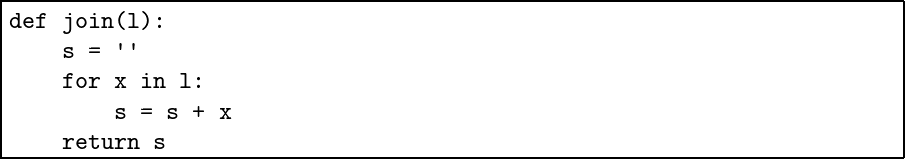Here is the result: Python >>> l = list('tumultuous') >>> l ['t', 'u', 'm', 'u', 'l', 't', 'u', 'o', 'u', 's'] >>> join(l) 'tumultuous' As you might suspect, there is a built-in join function: it is, somewhat counterintuitively, a method on strings. We specify the empty string and we see this: Python >>> l = list('tumultuous') >>> ''.join(l) 'tumultuous' If we specify a different string, it will be used to glue the letters together instead: Python >>> ' '.join(l) 't u m u l t u o u s' Another method on strings is split, which splits a given string into a list of strings, one for each word in the original: Python >>> s = ' Once upon a time ' >>> s.split() ['Once', 'upon', 'a', 'time'] As you can see, multiple spaces are considered the same as a single space, and spaces at the beginning and end are ignored. ### Finding strings in other strings The find method gives the index of the first position a string appears in another: Python >>> s = 'Once upon a time' >>> s.find('upon') 5 >>> s.find('not there') -1 In one of the questions, you will be asked to write a similar function yourself, from scratch. Of course, we can use indices and slices on strings too: Python >>> s = 'Once upon a time' >>> s >>> 'O' >>> s[:4] 'Once' >>> s[:-4] 'Once upon a ' >>> s[-4:] 'time' And so there is no need to convert a string to a list to take advantage of the useful slicing constructs. We can combine these two new techniques to isolate the first sentence in a string by removing anything which follows: Python >>> s = 'The first sentence. And the second...' >>> pos = s.find('.') >>> pos 18 >>> s[:pos + 1] 'The first sentence.' Of course, in practice we would need to check that find does not return -1. What would happen if it did? ### Sorting Now, we leave strings and return to lists. We often need to sort a list into increasing order prior to further processing. This can be achieved with the sort method: Python >>> l = [1, 2, 3, 2, 1, 3, 2] >>> l.sort() >>> l [1, 1, 2, 2, 2, 3, 3] The list is sorted in-place. The sorted function, on the other hand, returns a new, sorted version of the list, leaving the original list alone. Python >>> l = [1, 2, 3, 2, 1, 3, 2] >>> sorted(l) [1, 1, 2, 2, 2, 3, 3] >>> l [1, 2, 3, 2, 1, 3, 2] This is useful when we want to, for example, iterate over a list in sorted order but leave the original data intact for later use. ### Two useful functions: map and filter There are two built-in functions for producing lists by modifying other lists. The first is map which applies a function to each element of a list: Python >>> l = [1, 2, 3, 4, 5] >>> def square(x): return x * x ... >>> list(map(square, l)) [1, 4, 9, 16, 25] We must use list to retrieve the result. We shall discuss why in a moment. The second useful function is filter which can be used to select only such elements of a list for which a given function returns True: Python >>> l = [1, 2, 3, 4, 5] >>> def even(x): return x % 2 == 0 ... >>> list(filter(even, l)) [2, 4] You can imagine how these functions can be used instead of for loops, leading to shorter and easier to understand programs. As programmers, we spend a lot of our time reading programs we have already written (or reading programs written by others), compared with the time we spend writing new ones, so such ease of understanding is very important. ### Iterators We have just written this fragment, making use of map: Python >>> l = [1, 2, 3, 4, 5] >>> def square(x): return x * x ... >>> list(map(square, l)) [1, 4, 9, 16, 25] Why did we need to use list to convert the result of map into a list? It is because map returns an iterator not a list. An iterator is something which can be used to range over a data structure, but does not return a list – it returns items one by one. This means that the individual items are not created until they are needed. We can use a for loop over an iterator, without needing to make a list of it: Python >>> l = [1, 2, 3, 4, 5] >>> def square(x): return x * x ... >>> for x in map(square, l): ... print(x) ... 1 4 9 16 25 Another example of a function returning an iterator is Python’s reversed: Python >>> reversed([1, 4, 3, 2]) <list_reverseiterator object at 0x7fd45aa03dc0> >>> list(reversed([1, 4, 3, 2])) [2, 3, 4, 1] If we use reversed in a for loop, we would not notice that it did not return a list, but an iterator. Many built-in functions in Python operate over any iterable structure, not just lists: for example, sum calculates the sum of any such structure containing numbers. ### List comprehensions Instead of producing one list from another, or producing it manually by repeated use of append or insert, we can also build a list from scratch using a list comprehension. For example: Python >>> [x * x for x in range(10)] [0, 1, 4, 9, 16, 25, 36, 49, 64, 81] >>> [str(x) for x in range(10)] ['0', '1', '2', '3', '4', '5', '6', '7', '8', '9'] >>> [x % 2 == 0 for x in range(10)] [True, False, True, False, True, False, True, False, True, False] We can also provide a filter inside the list comprehension by adding an if at the end. Here are some cubes which are also even: Python >>> [x * x * x for x in range(20) if (x * x * x) % 2 == 0] [0, 8, 64, 216, 512, 1000, 1728, 2744, 4096, 5832] Such comprehensions provide a concise and readable way to produce lists of items meeting certain criteria, without having to iterate over them with a for loop. ## Common problems The strange formulation of the join mechanism, as a method on the string which is being used to glue the other together, can lead to confusion. Consider, for example, the following two strings: Python >>> x = 'marginal' >>> y = ' ' We intend to write the following: Python >>> y.join(x) 'm a r g i n a l' But if we get the strings in the wrong order, the operation still succeeds, but the result is not what we wanted: Python >>> x.join(y) ' ' The find method on strings has a way of signalling failure which we have not seen before: instead of returning an error, it returns normally but with an answer of -1. We must check for this, otherwise the -1 may be used unwittingly by the rest of the program without errors, for example in a slice: Python >>> 'Once'[-1] 'e' ## Summary We have learned about some of the connections between strings and lists, two kinds of ordered data structure. We have manipulated strings by splitting and joining them, and found strings within one another. We have introduced the important topic of sorting. We have seen maps and filters, two powerful mechanisms for processing lists. We have shown how iterators can simplify list-heavy programs. Finally, we have looked at list comprehensions, a way of combining one or more of these mechanisms together. ## Questions 1. Use the sort method to build a function which returns an alphabetically sorted list of all the words in a given sentence. 2. Use sorted to write a similar function. 3. Use a sorting method to make our histogram function from question 7 of the previous chapter produce the histogram sorted in alphabetical order. 4. Write a function to remove spaces from the beginning and end of a string representing a sentence by converting the string to a list of its letters, processing it, and converting it back to a single string. You might find the built-in reverse method on lists useful, or another list-reversal mechanism. 5. Can you find a simpler way to perform this task, using a built-in method described in this chapter? 6. Write a function clip which, given an integer, clips it to the range 1…10 so that integers bigger than 10 round down to 10, and those smaller than 1 round up to 1. Write another function clip_list which uses this first function together with map to apply this clipping to a whole list of integers. 7. Write a function to detect if a given string is palindromic (i.e. equals its own reverse). Now use filter to write a function which takes a list of strings and returns only those which are palindromic. Then write a function to return a list of the numbers in a given range which are palindromic, for example 1331. 8. Rewrite your clip_list example from question 6 in the form of a list comprehension. 9. Similarly, rewrite your palindromic number detector from question 7 in the form of a list comprehension. # 6. Prettier Printing We have been printing out information using the built-in print function. Sometimes, however, we have had to concatenate many little strings with + to insert into sentences the values we want to print, or use inconvenient extra parameters like end=” to prevent default behaviour giving an undesirable result. In this chapter, we will review the print function, and then explore a better method of printing with Python. ### Recalling the print function The print function takes a value. If the value is not a string, it converts it to a string with str. Then, it prints it to the screen and moves one line down by printing a newline character: Python >>> print('entrance') entrance >>> print(1) 1 >>> print([1, 2, 3]) [1, 2, 3] We have sometimes suppressed the newline by using an end argument: Python >>> print('entrance', end='') entrance>>> ### Printing with separators We can supply more or fewer arguments to the print function: Python >>> print() >>> print('one', 'two', 'three') one two three We see that print with no arguments just prints a newline. Supplying multiple arguments will print them all out, separated by spaces. We can change the separator: Python >>> print('one', 'two', 'three', sep='-') one-two-three ### Easier printing with format strings The print function is useful, but becomes rather clumsy when we are doing more complicated formatting. Python provides more advanced printing through what are called format strings. Here is a function to print the minimum and maximum items in a list of numbers as we might write it traditionally: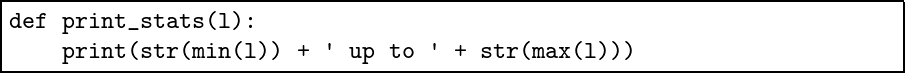(We wrote our own minimum and maximum functions earlier, but they are in fact built in to Python). Here it is in use: Python >>> print_stats([2, 3, 5, 7, 11, 13, 17, 19, 23, 29]) 2 up to 29 Now, the same function using a format string: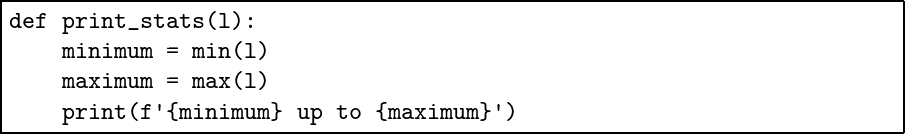There are two things to notice. First, the use of f’ to begin a string instead of just ’. This denotes a format string. Second, the sections inside the format string which are demarcated with curly braces {…}. The variable names in these will be substituted for the values of those variables. In fact, we can put whole expressions in the curly braces, simplifying further: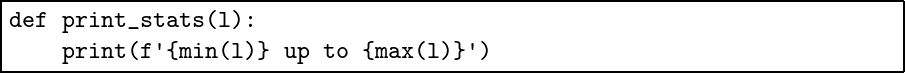Even in this simple example, we can see that it is rather easier to read our program when written with format strings, when compared with the repeated concatenation in the original. Consider a function to print out a table of powers (the ** operator raises a number to a power):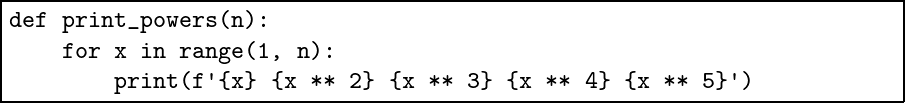Much like our times table in chapter 3, the columns are not lined up: Python >>> print_powers() 1 1 1 1 1 2 4 8 16 32 3 9 27 81 243 4 16 64 256 1024 5 25 125 625 3125 6 36 216 1296 7776 7 49 343 2401 16807 8 64 512 4096 32768 9 81 729 6561 59049 Format strings can do this for us automatically, with the addition of a format specifier within the curly braces. We add :5d at the end of each one. The 5 is for the column width, and d for decimal integer – the number will be right-justified in the column.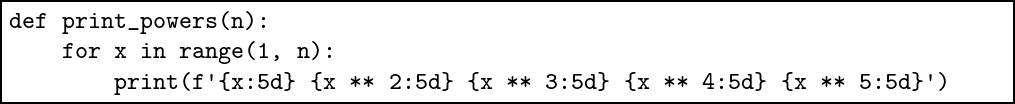Here is the result: Python >>> print_powers() 1 1 1 1 1 2 4 8 16 32 3 9 27 81 243 4 16 64 256 1024 5 25 125 625 3125 6 36 216 1296 7776 7 49 343 2401 16807 8 64 512 4096 32768 9 81 729 6561 59049 ### Printing to a file Instead of printing to the screen, we can print to a file by adding a file argument to the print function: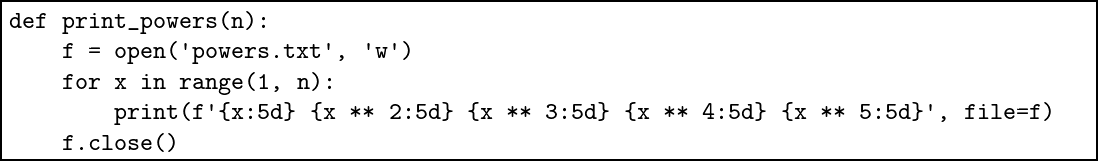The function here opens the new file ’powers.txt’ for writing (hence ’w’). We then supply the file argument to the print function. Afterward, we must be sure to close the file using the close method on the file f. A cleaner method is to use the with … as structure: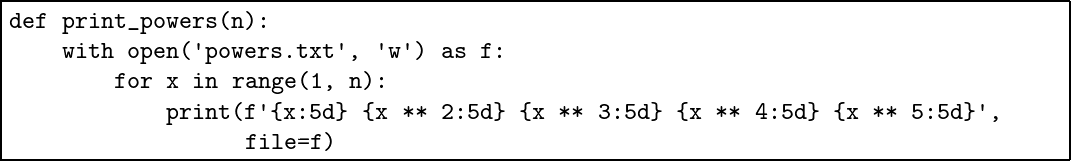The file will be closed automatically once the part of the program indented further to the right than the with is complete, so there is no need for us to close it explicitly. In the questions, we will use format strings to create some files of our own. ## Common problems We must remember to use the f prefix to our strings when using format strings, or we get the wrong result: Python >>> p = 15 >>> q = 12 >>> print('Total is {p + q}') Total is {p + q} Here is what it should look like: Python >>> print(f'Total is {p + q}') Total is 27 Quotation marks can end a format string, even when they are with the {} braces: Python >>> def two(x): return x + x ... >>> print(f'Twice is {two('twice')}') File "<stdin>", line 1 print(f'Twice is {two('twice')}') ^ SyntaxError: invalid syntax The solution is to use double quotation marks instead: Python >>> print(f"Twice is {two('twice')}") Twice is twicetwice Comments cannot appear inside braces: Python >>> print(f'This is the result: {result #update later}') File "<stdin>", line 1 SyntaxError: f-string expression part cannot include '#' Finally, when opening a new file for output with the withas …construct, remember that we must specify ’w’. Python >>> open('output.txt') Traceback (most recent call last): File "<stdin>", line 1, in <module> FileNotFoundError: [Errno 2] No such file or directory: 'output.txt' >>> open('output.txt', 'w') ## Summary We have expanded our knowledge of the built-in print function beyond the simple uses we encountered before. We learned about the powerful notion of format strings, and how to use them to shorten and simplify our code. Finally we printed to files, so our programs can now have effects persist even when we close Python. ## Questions 1. We can print a list like [1, 2, 3] easily using the print function. Imagine, though, that the print function could not work on lists. Write your own print_list function which uses simple print calls to print the individual elements, but adds the square brackets, commas and spaces itself. Do so without using format strings. 2. Now rewrite your function using format strings. Which is easier to read and write? 3. The method rjust on strings will right-justify them to the given width. Python >>> '2'.rjust(5) ' 2' Use this method to rewrite our print_powers function without format strings, but still with properly lined-up columns. 4. The method zfill on a string, given a number, will pad the string with zeroes to that width. For example, ’435’.zfill(8) will produce 00000435. Modify your previous answer to use this function to print our table of powers with uniform column widths padded by zeroes. 5. Write a program which asks the user to type in a list of names, one per line, like Mr James Smith, and writes them to a given file, again one per line, in the form Smith, John, Mr. 6. Rewrite the function from the previous question using format strings, if you did not use them the first time. 7. Use the find function introduced the previous chapter to write a program which prints the positions at which a given word is found in each of given list of sentences. For example, consider this list: ['Three pounds of self-raising flour', 'Two pounds of plain flour', 'Six ounces of butter'] Your function, given this list and the string ’pound’, should print: pound found at position 6 in sentence 1 pound found at position 4 in sentence 2 pound not found in sentence 3 8. Modify your answer to question 7 to print the information to a file with a given name. # 7. Arranging Things We have already seen how to combine values into a list. Lists are ordered, and mutable (we may alter elements, or insert or delete them). Sometimes we would like compound values with different properties. In this chapter, we look at three such structures: tuples, dictionaries, and sets. We will see how to choose the appropriate structure for the appropriate task: a program and its data structures are intimately linked. ### Tuples A tuple is a fixed-length collection of values, allowing the whole structure to be given a name and to be passed around just like we pass around any other value. There are two differences with lists: tuples are of fixed length, and their elements may not be altered. Here are some tuples: Python >>> t = (1, 'one') >>> t2 = (1, (1, 2), (1, 2, 3)) We now have two tuples: the first, t, of length 2, and the second, t2, of length 3, containing within it other tuples. We can take the tuples apart by assigning names. This is called unpacking: Python >>> a, b = t >>> a 1 >>> b 'one' >>> c, d, e = t2 >>> c 1 >>> d (1, 2) >>> e (1, 2, 3) We can pass a tuple to a function as usual, then unpack the values. For example, here is function to add two numbers passed to it as a single tuple of length 2: Python >>> def f(x): ... a, b = x ... return a + b ... >>> pair = (1, 2) >>> f(pair) 3 >>> f((1, 2)) 3 >>> f(1, 2) Traceback (most recent call last): File "<stdin>", line 1, in <module> TypeError: f() takes 1 positional argument but 2 were given We can also select items from a tuple using indexing and slicing: Python >>> t2 = (1, (1, 2), (1, 2, 3)) >>> t2 1 >>> t2[::-1] ((1, 2, 3), (1, 2), 1) Of course, to do this without knowing the length of the tuple might sometimes be difficult. We can use the usual len function: Python >>> t2 = (1, (1, 2), (1, 2, 3)) >>> len(t2) 3 >>> len(t2) 2 Tuples are immutable – unlike with lists, we cannot change their elements. Python >>> x = (1, 2) >>> x = 3 Traceback (most recent call last): File "<stdin>", line 1, in <module> TypeError: 'tuple' object does not support item assignment Of course, if a tuple contains an mutable value such as a list, we can change parts of that inside value: Python >>> l = [1, 2, 3] >>> t = (l, l) >>> l = 4 >>> t ([4, 2, 3], [4, 2, 3]) ### Dictionaries Many programs make use of a structure known as a dictionary. A real dictionary is used for associating definitions with words; we use “dictionary” more generally to mean associating some unique keys (like words) with values (like definitions). For example, we might like to store the following information about the number of people living in each house in a road: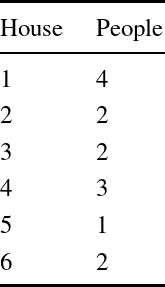We could represent this using a list of pairs represented as tuples. But then we would have to write various functions for looking up or replacing entries ourselves. Python provides a special type for dictionaries, which preserves automatically the property that every key has only one value associated with it. Let us start with an empty dictionary, which is written {}, and add and update some entries: Python >>> d = {} >>> d = 4 >>> d {1: 4} >>> d = 2 >>> d = 2 >>> d = 3 >>> d = 1 >>> d = 2 >>> d {1: 4, 2: 2, 3: 2, 4: 3, 5: 1, 6: 2} >>> d = 8 >>> d {1: 4, 2: 2, 3: 2, 4: 3, 5: 1, 6: 8} We could, of course, write the whole thing in one go: Python >>> d = {1: 4, 2: 2, 3: 2, 4: 3, 5: 1, 6: 2} We can see that dictionaries are unordered: Python >>> {1: 4, 2: 2} == {2: 2, 1: 4} True Keys in a dictionary must be immutable. For example, we cannot use a list as a key. We can use the usual tests in and not in to check if a dictionary has a value for a given key: Python >>> d = {1: 4, 2: 2, 3: 2, 4: 3, 5: 1, 6: 2} >>> 1 in d True >>> 10 in d False >>> 10 not in d True Finally, deletion is performed with the usual del statement, providing the key only: Python >>> d = {1: 4, 2: 2, 3: 2, 4: 3, 5: 1, 6: 2} >>> del d >>> d {1: 4, 3: 2, 4: 3, 5: 1, 6: 2} There is an error if the key is not in the dictionary: >>> del d Traceback (most recent call last): File "<stdin>", line 1, in <module> KeyError: 7 ### Iterating over dictionaries How might we iterate over the dictionary entries? We use a for loop, but we specify two names: one for the key, and one for the value, and use the items method: Python >>> for k, v in d.items(): ... print(f'{k} is mapped to {v}') ... 1 is mapped to 4 2 is mapped to 2 3 is mapped to 2 4 is mapped to 3 5 is mapped to 1 6 is mapped to 2 Alternatively, we can use an ordinary for loop, and simply look the value up: Python >>> for k in d: ... print(f'{k} is mapped to {d[k]}') ... 1 is mapped to 4 2 is mapped to 2 3 is mapped to 2 4 is mapped to 3 5 is mapped to 1 6 is mapped to 2 Should we have key-value pairs already, we can turn a list of them into a dictionary using the dict function: Python >>> dict([(1, 'one'), (2, 'two'), (3, 'three')]) {1: 'one', 2: 'two', 3: 'three'} >>> dict([(1, 'ONE'), (1, 'one'), (2, 'two'), (3, 'three')]) {1: 'one', 2: 'two', 3: 'three'} Notice that the entry (1, ’ONE’) is overwritten, since the entries are added in order. ### Sets In the questions to chapter 4 we wrote a function setify to remove duplicate items from a list. Python has a built-in type for sets: they are just like dictionaries, but with no values. Python >>> s = {1, 2, 3} >>> s2 = set([1, 2, 3, 2, 1]) >>> s2 {1, 2, 3} >>> s3 = set('qwertyuiop') >>> s3 {'w', 'p', 'r', 'e', 'i', 'q', 'o', 'u', 'y', 't'} >>> empty_set = set() >>> empty_set set() Note that the empty set is built by, and printed as set(). This is to distinguish it from the empty dictionary {}. We can use the usual in and not in tests: Python >>> s = set('qwertyuiop') >>> 'e' in s True >>> 'z' not in s True To add an item to a set, we use the add method: Python >>> s = set([1, 2, 3, 4, 4, 5]) >>> s {1, 2, 3, 4, 5} >>> s.add(7) >>> s {1, 2, 3, 4, 5, 7} To remove an item from a set, we use the remove method: Python >>> s = set([1, 2, 3, 4, 4, 5]) >>> s {1, 2, 3, 4, 5} >>> s.remove(4) >>> s {1, 2, 3, 5} There is an error if the item to remove is not in the set. Finally, there are four operations for manipulating pairs of sets: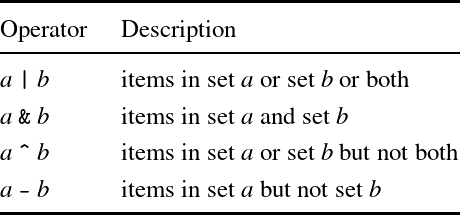For example: Python >>> a = {1, 2, 3, 4} >>> b = {1, 2, 5, 6} >>> a | b {1, 2, 3, 4, 5, 6} >>> a & b {1, 2} >>> a ^ b {3, 4, 5, 6} >>> a - b {3, 4} Set operations are useful when we need information from two sources to select what to do next, or which data to operate on next. ## Common problems One must take care to distinguish between parentheses used for multiple arguments to a function, and parentheses used for building a tuple: Python >>> def f(a, b): return a + b ... >>> x = (1, 2) >>> f(x) Traceback (most recent call last): File "<stdin>", line 1, in <module> TypeError: f() missing 1 required positional argument: 'b' Tuple unpacking must be explicit. For example, imagine a function we wished to use by passing two arguments, a number and a pair of numbers: Python >>> f(1, (2, 3)) 7 We might like to write this, but Python will not let us: Python >>> def f(a, (b, c)): return a + b * c File "<stdin>", line 1 def f(a, (b, c)): return a + b * c ^ SyntaxError: invalid syntax Instead, we must explicitly unpack the tuple: Python >>> def f(a, pair): b, c = pair return a + b * c Dictionaries exhibit, of course, the usual lookup errors when a key is not found: Python >>> d = {1 : 2, 2 : 3} >>> d Traceback (most recent call last): File "<stdin>", line 1, in <module> KeyError: 3 More subtly, it is important to remember that in and not in refer to the keys present in a dictionary, not the values: Python >>> d = {1 : 2, 2 : 3} >>> 3 in d False ## Summary We have looked at some new data structures: tuples for holding two or more items; and dictionaries which assign keys to values. We concluded with sets, which can be used to store information without duplicates, and quickly to test for membership. We have seen how to build sets from strings. For a long time now, we have been saying that we would address detection and recovery from errors. In the next chapter, we do just that. ## Questions 1. We can swap the values of variables a and b by using a temporary variable t: Python >>> a = 1 >>> b = 2 >>> t = a >>> a = b >>> b = t >>> a 2 >>> b 1 Show how to use a tuple to achieve the same result. 2. Write a function unzip which, given a dictionary, returns a pair of lists, the first containing the keys and the second the corresponding values. 3. The opposite function zip, combined with the dict function we have already described, can be used to build a dictionary from two lists: one of all the keys, and one of all the values. Python >>> dict(zip([1, 2], ['one', 'two'])) {1: 'one', 2: 'two'} Write a function to replace both zip and dict in this circumstance. 4. Write the function union(a, b) which forms the union of two dictionaries. The union of two dictionaries is the dictionary containing all the entries in one or other or both. In the case that a key is contained in both dictionaries, the value in the first should be preferred. 5. The following, flawed function is intended to remove all items equal to zero from a list: Python >>> def remove_zeroes(l): ... for x in range(0, len(l)): ... if l[x] == 0: del l[x] ... >>> >>> l = [1, 0, 0, 0, 1] >>> remove_zeroes(l) Traceback (most recent call last): File "<stdin>", line 1, in <module> File "<stdin>", line 3, in remove_zeroes IndexError: list index out of range >>> l [1, 0, 1] Why does it fail? Write a correct version. 6. We can write dictionary comprehensions, much like list comprehensions. For example: Python >>> {n: n ** 2 for n in range(10)} {0: 0, 1: 1, 2: 4, 3: 9, 4: 16, 5: 25, 6: 36, 7: 49, 8: 64, 9: 81} >>> {n: n ** 2 for n in range(10) if n ** 2 % 2 == 0} {0: 0, 2: 4, 4: 16, 6: 36, 8: 64} Write a dictionary comprehension to ‘reverse’ a dictionary, that is to make the keys in the original values and the values in the original keys. Why might the new dictionary have a different size from the original? 7. Use sets to write a function which returns the ‘letter set’ of a list of words. That is to say, the list of all letters used in those words. Now write a function to return a set of all letters not used by them. 8. Imagine Python did not have built-in support for sets. Show how we could use dictionaries to represent sets. Write the four set operations | - ^ & for this new representation of sets. 9. Write the set operation & using set comprehensions. Set comprehensions look a little like the dictionary comprehensions of question 6. We can use two for sections to cycle over all pairs of set members i.e. for x in a for y in b 10. Write a function to add the numbers in a tuple. For example, sum_all(1, (1, 2), 3) should yield 7. You will need to distinguish between integers and tuples by using the test type(x) == int, which is True if the type of x is int. # 8. When Things Go Wrong As we have seen, sometimes programs fail to produce a result, ending instead in an error. Sometimes, we do not even get that far – Python rejects our program when we type it in, before we have a chance to run it. Sometimes the error is in our program itself, the programmer’s fault. Sometimes it is a problem with unexpected input from the user, or the absence of an expected file. In this chapter, we look at strategies for detecting, coping with, and recovering from these various types of error. ### When there is no result We will begin by looking at Python’s mechanism for dealing with null results. You might have noticed that if we forget the return keyword, we see this: Python >>> def f(a, b): a + b ... >>> f(1, 2) >>> It looks as if nothing is returned. In fact, the result is a special value called None: Python >>> f(1, 2) is None True >>> None >>> (We use the is operator here instead of ==, for reasons beyond the scope of this book.) Note that None has no printed representation here unless we explicitly use print, or if it appears in a compound structure: Python >>> print(f(1, 2)) None >>> def g(a): ... if a > 0: ... return a ... else: ... pass ... >>> list(map(g, [-1, 0, 1, 2, 3])) [None, None, 1, 2, 3] The None value has a type. In fact, it is the only value of that type: Python >>> type(None) <class 'NoneType'> Some operations which raise errors have equivalent versions which instead return None on an error. For example, looking up a key in a dictionary with the get method instead of with ordinary indexing returns None: Python >>> d = {1: 'one', 2: 'two', 3: 'three'} >>> d Traceback (most recent call last): File "<stdin>", line 1, in <module> KeyError: 0 >>> d.get(0) >>> v = d.get(0) >>> print(v) None So, we could write a function to look up a list of given keys in a dictionary, returning a list of only the values for which lookup succeeds, and ignoring those for which it fails: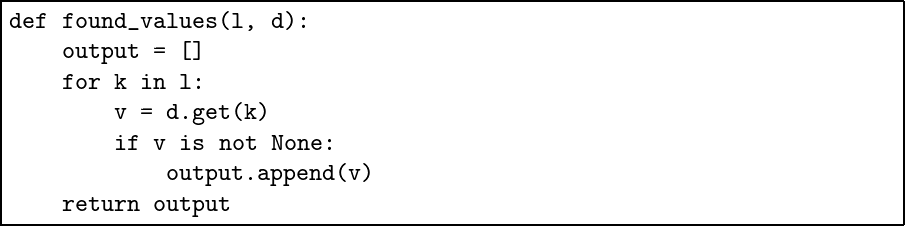(We can write is not as well as is). For example: Python >>> found_values([1, 2, 3], {1: 'one', 2: 'two'}) ['one', 'two'] ### Exceptions Python has a mechanism for representing, detecting, and responding to exceptional situations. That mechanism is known as an exception. We have just seen an example: Python >>> d = {1: 'one', 2: 'two', 3: 'three'} >>> d Traceback (most recent call last): File "<stdin>", line 1, in <module> KeyError: 0 The exception here is KeyError, and it carries along with it the number 0 so we know which key could not be found. Let us write a dictionary lookup function which prints our own message and returns -1 if the lookup fails: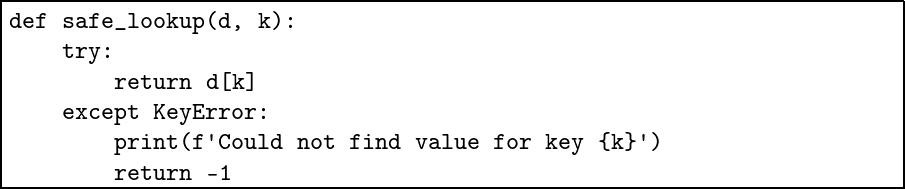There are two new words here: try and except. The statements after try will be attempted. If they succeed, the function returns as normal. If they fail with KeyError, control transfers to the except section. Here is an example failing call: Python >>> safe_lookup({1: 'one', 2: 'two', 3: 'three'}, 0) Could not find value for key 0 -1 By this exception mechanism, we can handle exceptional circumstances without stopping the program, or terminate the program early, but in a controlled manner. ### Standard exceptions Here are some of Python’s standard exceptions: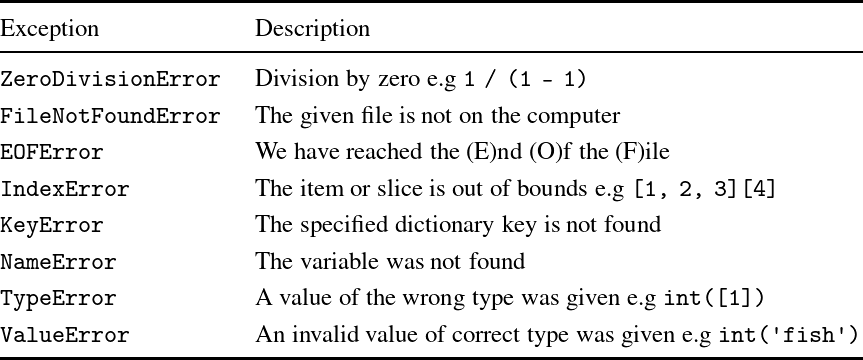Here is an example of the NameError exception: Python >>> def add3(x, y): return x + y + z ... >>> add3(1, 2) Traceback (most recent call last): File "<stdin>", line 1, in <module> File "<stdin>", line 1, in add3 NameError: name 'z' is not defined >>> z = 10 >>> add3(1, 2) 13 Notice that z does not have to be defined after the definition of add3 – it may be supplied afterward. This is rather bad practice through, of course. ### Raising exceptions ourselves As well as handling the standard exceptions, we can raise them ourself with the raise construct. Here is a function to build a list of repeated elements: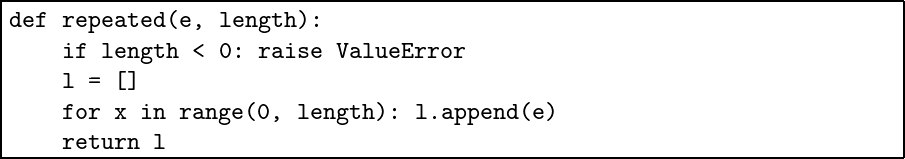This function raises ValueError if asked to create a list of negative length. For example: Python >>> repeated(1, 10) [1, 1, 1, 1, 1, 1, 1, 1, 1, 1] >>> repeated(1, -10) Traceback (most recent call last): File "<stdin>", line 1, in <module> File "<stdin>", line 2, in repeated ValueError We can give a name to an exception as we catch it, allowing us to use raise to re-raise the exception: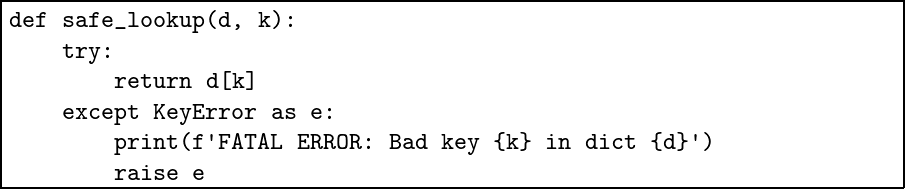Here, we have decided that a bad key is a fatal error, but we wish to provide some debugging information including the key and dictionary before the program ends. ### Catching any exception We can catch any exception by using except Exception: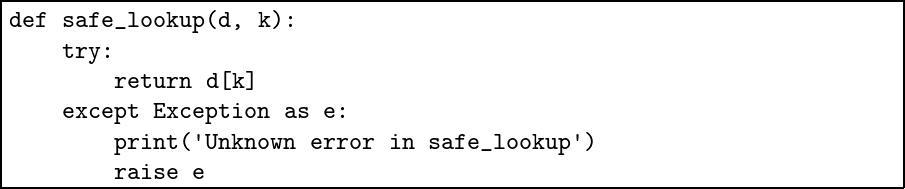We would only normally do this to gather up any un-handled exceptions in a large program, and report them cleanly before exiting. Otherwise it is always best to specify which exception we expect to have to handle. ### Keeping exceptions small We should try to keep the part between try and except as small as possible, so it is clear which statement or statements might fail to complete. We can do this using an optional else part: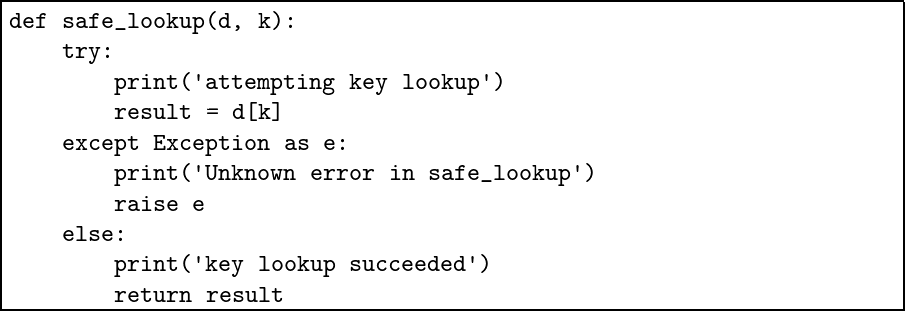Notice that the variable result is available in the else portion. We can now rewrite, using exceptions, our guessing_game function from the questions to chapter 3. We wish to properly deal with the possibility that the input from the user is either not a number, resulting in a ValueError exception, or that the number is not in range. We will encapsulate this in the new get_guess function, using a fairly benign form of recursion: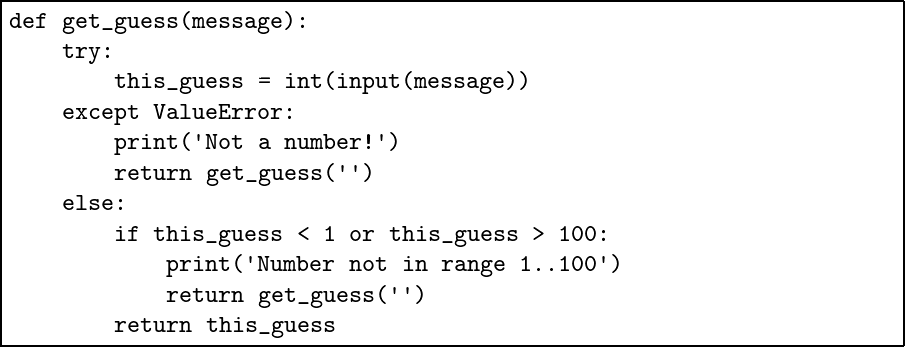We minimise the portion between try and except by including an else section. Now the error handling is confined to the get_guess function, and the main function is relatively simple: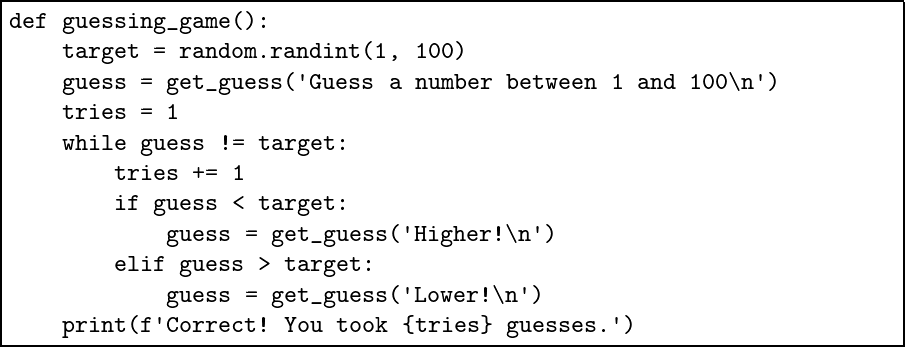Here is the full program: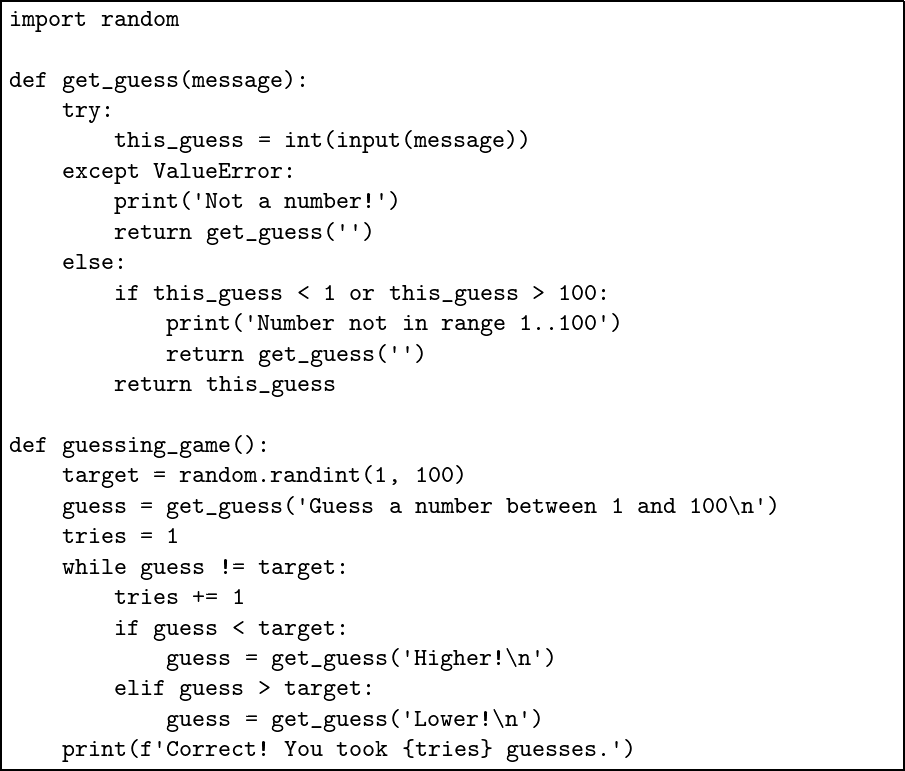## Common problems Being a language which evolved slowly, with no grand design, there is in Python little consistency. Some functions signal error by returning -1, some by returning None, some by raising exceptions. It is important to check the documentation, and make sure to include error handling in our programs at all appropriate points. Even if we have to exit the program on a particularly unusual error (e.g disk full), we can at least print a message. Taking care in this circumstances is crucial to building reliable programs, especially larger ones. ## Summary We have finally addressed the problem of how to deal with errors which occur when running our programs: to detect them, handle them, and recover from them. We have learned about the null result None and how to take advantage of it. We can now add exceptions to our toolbox, choosing between error avoidance and error detection as appropriate in each situation. In the next chapter, we return to the topic of file processing, writing some more complete programs. ## Questions 1. Write a function which, given a list of strings, such as [’1’, ’10’, ’ten’, ’tree’] returns their sum, ignoring anything which is not a number made of digits. 2. Rewrite your solution using map, filter, and sum, if you did not use them originally. 3. Use exceptions to write a safe_division function which returns 0 if asked to divide by zero. 4. Use exceptions to write a function to prune a dictionary: dict_take(a, b) should yield a new dictionary with keys and values drawn from dictionary b, but only if the key exists in dictionary a. 5. Write a function safe_union which builds the union of two dictionaries, but raises KeyError if there is a clash of keys. 6. Write a function add_exception to add value to a set, but which raises KeyError if the value already exists in the set. # 9. More with Files In chapter 6, we saw how to print to a file instead of to the screen. In this chapter, we will see how to read information from existing files. Then we will write programs to process data from files, and to edit files. ### Reading from files We shall consider the opening paragraph of Kafka’s “Metamorphosis”.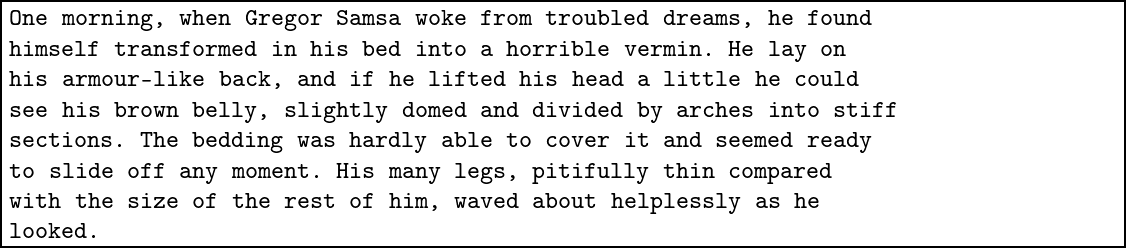There are newline characters at the end of each line, save for the last. You can cut and paste or type this into a text file to try these examples out. Here, it is saved as gregor.txt. Now, we can read the whole contents of the file into a string using ’r’ for reading mode: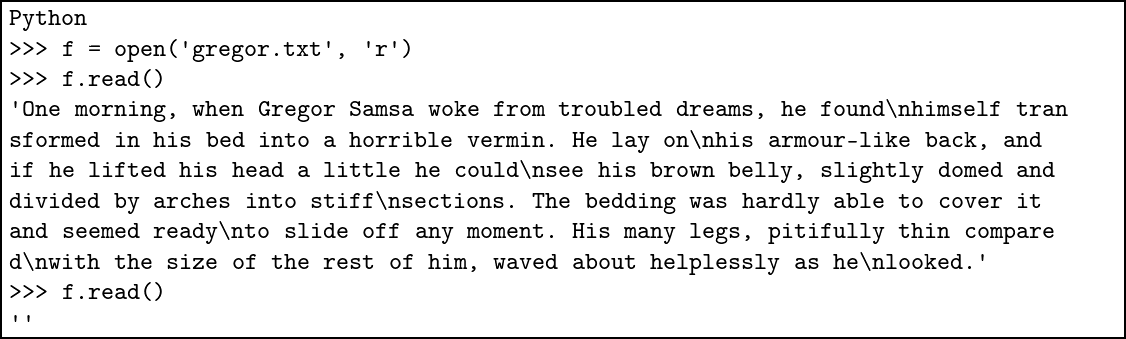This single string contains the \n newline characters, of course. If we call f.read() again, the result is the empty string. This is because there is nothing else left to read – the contents of the file has already been read and we are at the end of the file. ### Three ways to iterate over lines Instead of reading the whole file as one big string, we may read the lines in turn, by repeated use of the readline method: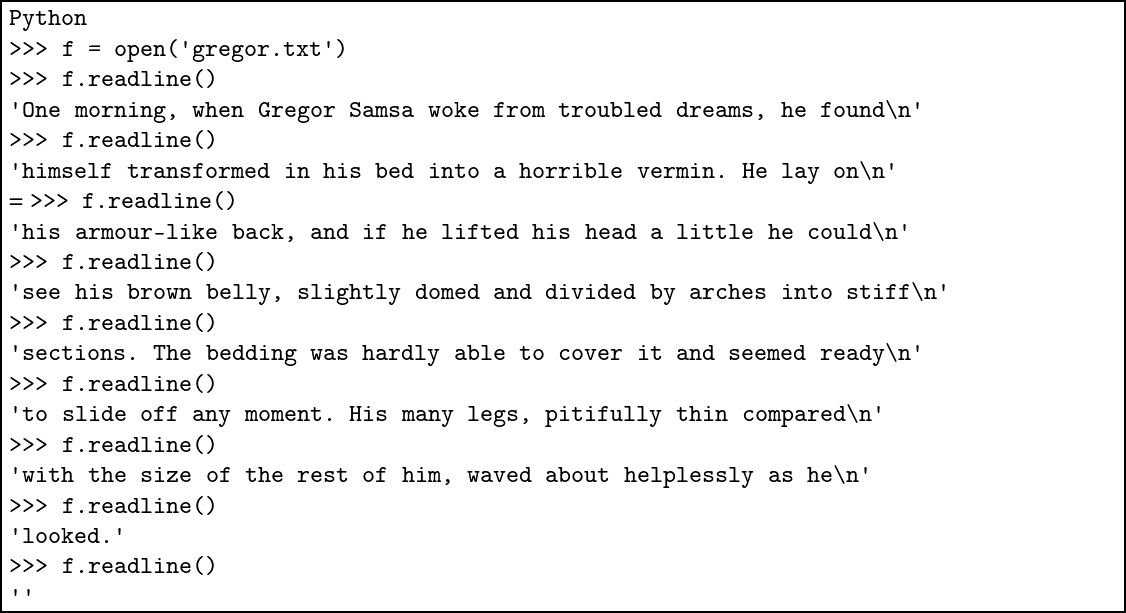Notice that we omit the ’r’ argument to the open function – it is the default. Again, we know that there is no more to read when the result is the empty string. We can, alternatively, iterate directly over the contents of the file with a for loop: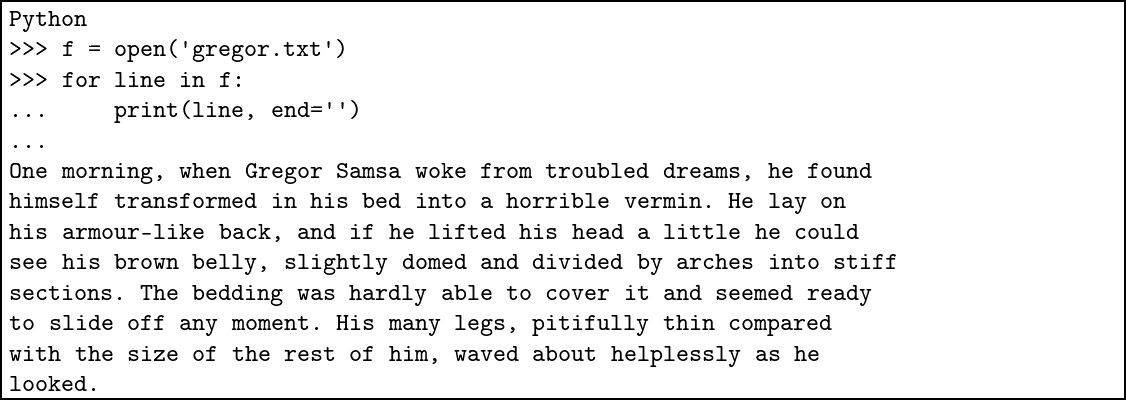Finally, we can use the list function to return a list of all the lines in the file in one go: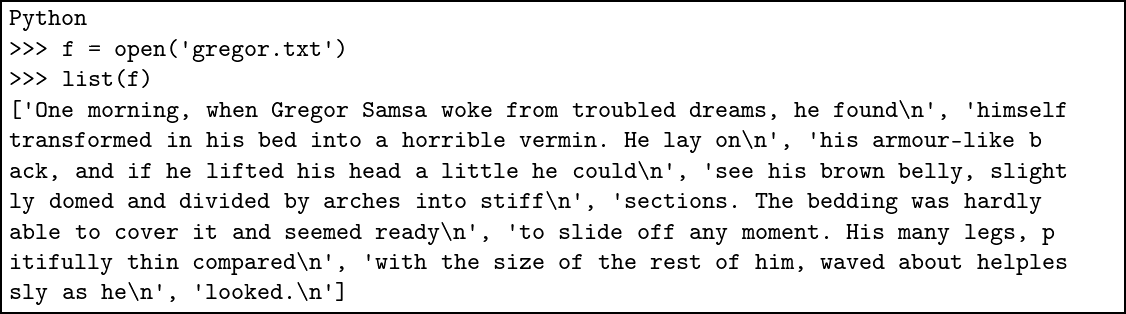### Example: reversing lines We can write a program to read all the lines from a file, and write them in reverse order to another file: Python >>> f = open('gregor.txt') >>> f_out = open('output.txt', 'w') >>> for x in reversed(list(f)): ... print(x, end='', file=f_out) ... >>> f.close() >>> f_out.close() Here is the contents of the output file: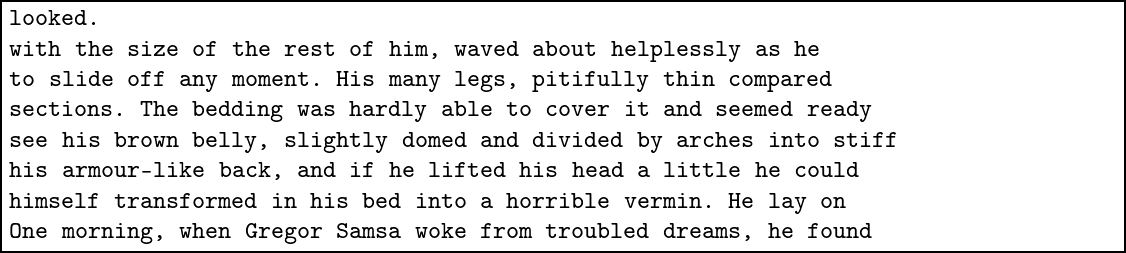We can use an extended version of the with … as structure we have already seen to prevent mistakes with matching up the opening and closing of files. Here is the same program in this simpler, safer, form: Python >>> with open('gregor.txt') as f, open('output.txt', 'w') as f_out: ... for x in reversed(list(f)): ... print(x, end='', file=f_out) ### Files and exceptions Not only does the with … as construct prevent double-closing of a file, but also prevents any attempt to read from a file which has already been closed: Python >>> f = open('gregor.txt') >>> f.close() >>> f.read() Traceback (most recent call last): File "<stdin>", line 1, in <module> ValueError: I/O operation on closed file. There are still, however, some exceptions we may need to handle, even when using with … as – for example, a missing file: Python >>> open('not_there.txt') Traceback (most recent call last): File "<stdin>", line 1, in <module> FileNotFoundError: [Errno 2] No such file or directory: 'not_there.txt' ### Example: text statistics Consider this program to return the number of lines, characters (letters or other symbols), words, and sentences in a given file: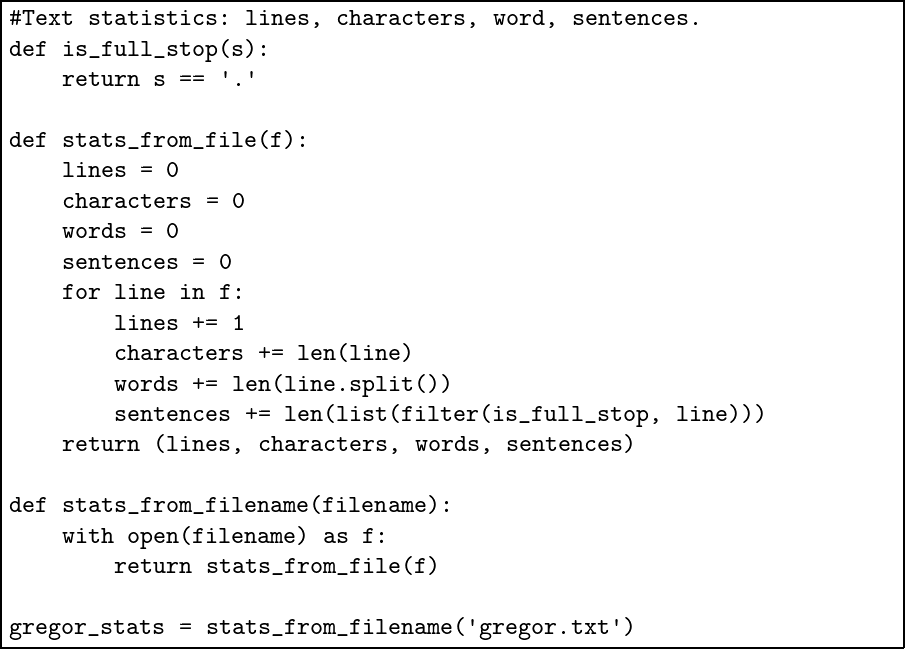Notice we can use filter directly on line without turning it into a list. Here is the result: Python >>> gregor_stats (8, 472, 85, 4) That is to say, 8 lines, 472 characters, 85 words, and 4 sentences. In the questions, you will be asked to extend this program to collect more statistics. ## Common problems In addition to situations which can lead to file-related exceptions, there are two more common issues which can occur when processing files. If we open a file which already exists, with the intention of writing to it, but we forget to open it in ’w’ mode, an exception occurs: Python >>> with open('exists.txt') as f: ... print('output', file=f) ... Traceback (most recent call last): File "<stdin>", line 2, in <module> io.UnsupportedOperation: not writable When printing lines from a file which we have read using, for example, readlines, it is important to remember that they will always have a \n newline at the end already. If we print them just using plain print we will see double spacing: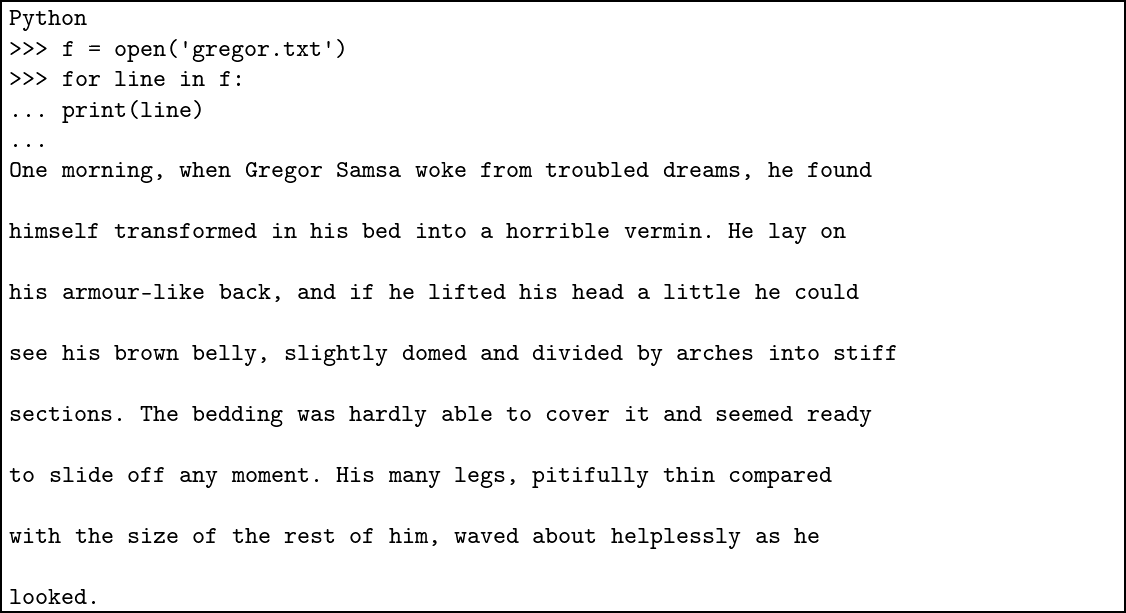## Summary We now know how to read from files as well as write to them. This means we can write file-processing programs, which read from one file, process the data in some way, and write to another. This is a significant class of useful programs. We made our programs cleaner and less error-prone by extending our use of the with … as construct. We learned how to iterate over the lines in the file, and so over the characters in each line, building our file statistics program. In some of the questions, you will be asked to extend this file statistics program in various ways. In the next chapter, we fill in another gap in our knowledge: the real numbers – that is to say the ones which are not whole numbers. ## Questions 1. We wrote a program to print out the contents of a file line-by-line: Python >>> f = open('gregor.txt') >>> for line in f: ... print(line, end='') Rewrite this program using the with … as construct. 2. Give a function to write a dictionary with integer keys and string values to a given file. For example, the dictionary {1: ’oak’, 2: ’ash’, 3: ’lime’} should produce the file: 1 oak 2 ash 3 lime 3. Now write a function to read such a dictionary back from file. Make sure to handle exceptions arising from incorrect data. There is a built-in method strip which removes spaces and newlines from either end of a string which may prove useful. 4. When we write to a file which already exists, its contents are overwritten. The file mode ’a’ allows information to be appended to a file instead. Use this to write a function which concatenates two files, writing the result to a third. 5. Write a function which reads a file containing multiple numbers, separated by spaces, on multiple lines, and calculates their total. 6. Write a function copy_file which, given two file names, reads the contents of the first, and writes it to the second. 7. Extend our text statistics to print a histogram of the frequencies of each letter in the file. You might remember we wrote a similar histogram program in the questions to chapter 4. 8. Extend it again to print a histogram of frequencies of words. How might punctuation and capital letters be dealt with? Hints: • There is a built-in method strip on strings which can be given an argument, a string containing the characters to be stripped. • The string string.punctuation following import string contains common punctuation characters. • The lower method on a string method converts it to lowercase. 9. Write a function to search for a given word in a given file, listing the line numbers and lines at which it appears. Use our lessons from the previous question to deal with punctuation. 10. Write a function top which prints the first five lines from a file, waiting for the user to press Enter for another five, and so on. # 10. The Other Numbers The only numbers we have considered until now have been the whole numbers, or integers. For a lot of programming tasks, they are sufficient. And, except for their limited range and the possibility of division by zero, they are easy to understand and use. However, we must now consider the real numbers. ### Introducing floating-point numbers It is clearly not possible to represent all numbers exactly – they might be irrational like π or e and have no finite numerical representation. For most uses, a representation called floating-point is suitable, and this is how Python’s real numbers are stored. Not all numbers can be represented exactly, but arithmetic operations are very quick. We can write a floating-point number by including a decimal point somewhere in it. For example 1.6 or 2. or 386.54123. Negative floating-point numbers are preceded by the - character just like negative integers. Here are some floating-point numbers in Python: Python >>> type(1.5) <class 'float'> >>> 6. 6.0 >>> -2.3456 -2.3456 >>> 1.0 + 2.5 * 3.0 8.5 >>> 1.0 / 1000.0 0.001 ### Mixing different kinds of number When we mix integers and floating-point numbers, Python will automatically convert the integer to a floating point so that the operation can work: Python >>> 1 + 2 * 3.0 7.0 Here the integer 2 is converted to the floating-point number 2.0 for the multiplication, which results in the floating-point result 6.0. Then the integer 1 must be similarly converted to a floating-point number to do the addition and produce the final result. The conversion only happens when the expression requires it: Python >>> type(1 + 2) <class 'int'> >>> type(1 + 2.0) <class 'float'> Sometimes an operation on two integers can produce a floating-point result, for example using the division operator: Python >>> 1 / 2 0.5 You can see now why we introduced addition, subtraction, and multiplication in chapter 1, but left out division. There is an integer division operator too: Python >>> 2 // 3 0 >>> 10 // 5 2 You can see that this operator calculates just the whole part. We already have the % modulus operator to calculate the remainder. ### Limits of range and precision Here is an example of the limits of precision in floating-point operations: Python >>> 3.123 - 3.0 0.12300000000000022 Very small or very large numbers are written using so-called scientific notation: Python >>> 1.0 / 100000.0 1e-05 >>> 30000. ** 10. 5.9049e+44 These are the numbers 1 × 10 − 5 and 5.9049 × 1044 respectively. We can find out the range of numbers available: Python >>> import sys >>> sys.float_info.max 1.7976931348623157e+308 >>> sys.float_info.min 2.2250738585072014e-308 Working with floating-point numbers requires care, and a comprehensive discussion is outside the scope of this book. These challenges exist in any programming language using the floating-point system. We will leave these complications for now – just be aware that they are lurking and must be confronted when writing robust numerical programs. ### Standard functions There are two built-in functions for converting between integers and floating-point numbers: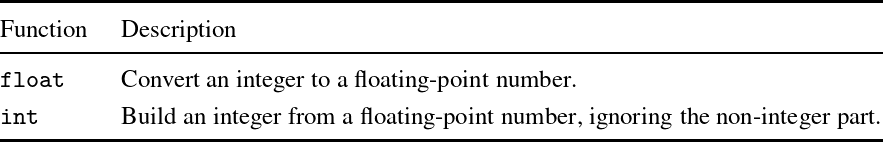Notice that int is not the expected rounding function: Python >>> float(2) 2.0 >>> int(2.3) 2 >>> int(2.8) 2 If we use import math, more functions are available: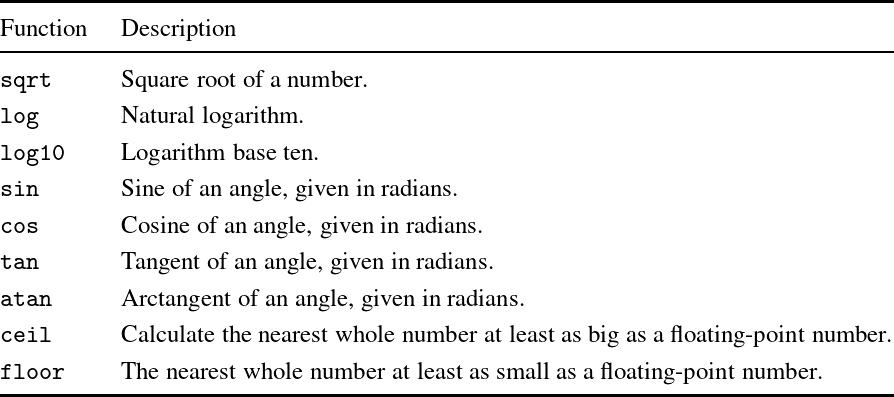For example, we can calculate: Python >>> import math >>> math.sqrt(3 * 3 + 4 * 4) 5.0 >>> math.sqrt(2) 1.4142135623730951 The ceiling and floor functions give us the rounding behaviour we expect: Python >>> math.ceil(2.3) 3 >>> math.floor(2.3) 2 >>> math.ceil(2.5) 3 Note that they return integers. But we can get back to floating-point easily, of course: Python >>> float(math.ceil(2.7)) 3.0 ### Example: vectors Let us write some functions with floating-point numbers. We will write some simple operations on vectors in two dimensions. We will represent a point as a pair of floating-point numbers such as (2.0, 3.0). We will represent a vector as a pair of floating-point numbers too. Now we can write a function to build a vector from one point to another, one to find the length of a vector, one to offset a point by a vector, and one to scale a vector to a given length: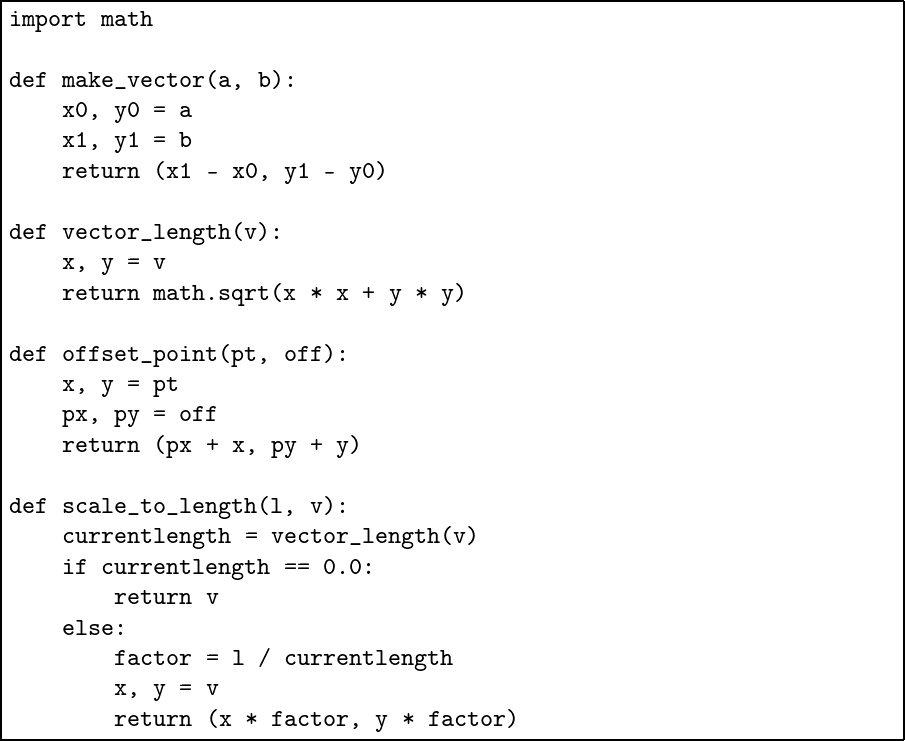Notice that we have to be careful about division by zero, just as with integers. We have used tuples for the points because it is easier to read this way – we could have passed each floating-point number as a separate argument instead, of course. Floating-point numbers are often essential, but must be used with caution. You will discover this when answering the questions for this chapter. Some of these questions require using the built-in functions listed in the table above. ## Common problems We should never use floating-point numbers to represent currency. For example, selling 145 items at$2.34:

Python
>>> 145 * 2.34
339.29999999999995

Instead, we can store the numbers as integer amounts of cents:

Python
>>> 145 * 234
33930

We only need consider dollars when formatting the number for printing, not when calculating with it.

Repeated calculations can lead to errors compounding. For example, repeated addition is not the same as multiplication when it comes to floating-point numbers:

Python
>>> x = 0.0
>>> for y in range(10):
...   x += 0.1
...
>>> x
0.9999999999999999
>>> 0.1 * 10
1.0

## Summary

We have filled in a gap in our knowledge of Python: how to use real numbers, or floating-point approximations of them. We have learned to be wary of them, and so to use them only when really needed. We looked at the wide range of standard functions for manipulating floating-point numbers, including the floor and ceil functions, and the int and float functions for converting between floating point numbers and integers.

In the next chapter we look at the Python Standard Library, Python’s collection of helpful modules, in more depth.

## Questions

1. Give a function which rounds a positive floating-point number to the nearest whole number, returning another floating-point number.

2. Write a function to find the point equidistant from two given points in two dimensions.

3. Write a function to separate a floating-point number into its whole and fractional parts. Return them as a tuple.

4. Write a function star which, given a floating-point number between zero and one, draws an asterisk to indicate the position. An argument of zero will result in an asterisk in column one, and an argument of one an asterisk in column fifty.

5. Now write a function plot which, given a function which takes and returns a real number, a start and end point, and a step size, uses star to draw a graph. For example we might see: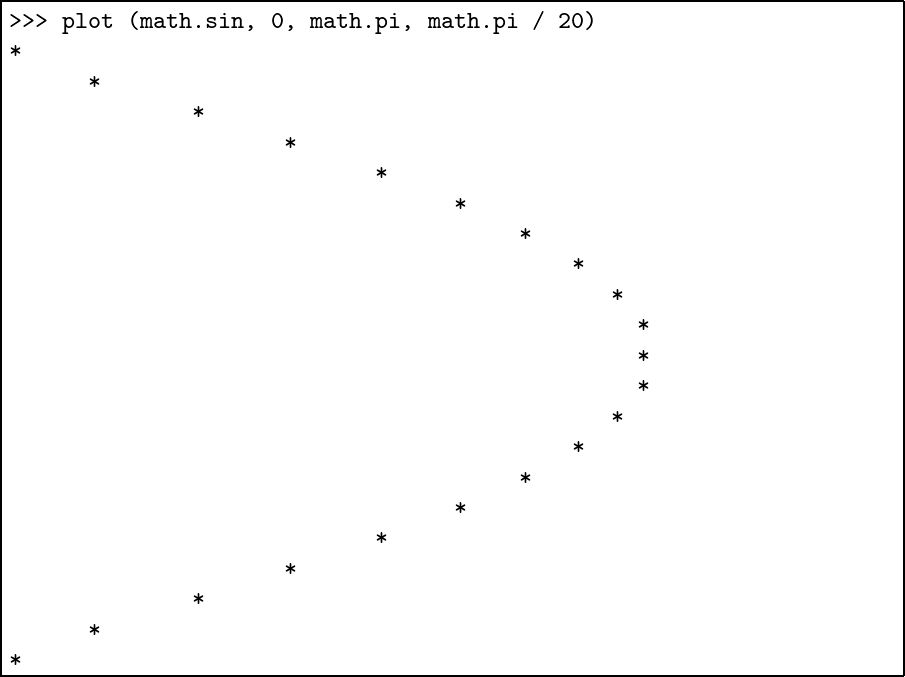Here, we have plotted the sine function on the range 0…π in steps of size π/20.

# 11. The Standard Library

We can divide the words and symbols we have been using to build Python programs into three kinds:

1. The language itself. For example, words like if and return. These also include operators like +.

2. Things which are not part of the language, but which are always available, such as input and map.

3. Things we had to ask for specifically by using import. These are extra modules supplied with Python, and called the Standard Library.

It is this last category which concerns us here.

### Python’s Standard Library

The Python Standard library is divided into modules, one for each area of functionality (in the next chapter, we will learn how to write our own modules). We have already seen how to use import statement to make available functions from a module. Here are the modules we have already used from the Standard Library: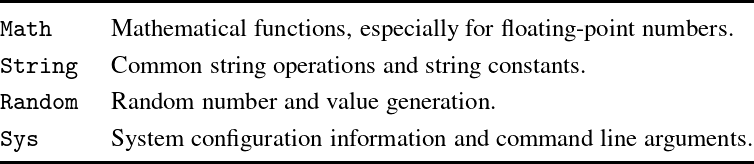### More about importing modules

Previously, we introduced the import construct. Let us review it now. We can use from … import to access definitions and functions from another module. As we know, the functions from a module can be used by putting a period (full stop) between the module name and the function. As an example, the perm function in the math module can be used like this:

Python
>>> import math
>>> math.perm(5, 2)
20

We can use from … import * to import all definitions from a script:

Python
>>> from math import *
>>> perm(5, 2)
20

We would not normally do this with Standard Library modules: names may clash with our own functions, leading to bugs. We can reduce this problem by importing only the functions we want:

Python
>>> from math import perm, factorial
>>> perm(5, 2)
20
>>> factorial(10)
3628800
>>> ceil(2.3)
Traceback (most recent call last):
File "<stdin>", line 1, in <module>
NameError: name 'ceil' is not defined

In any event, if we need to use a function with a long name several times, we can rename it ourselves:

Python
>>> import math
>>> fac = math.factorial
>>> fac(10)
3628800

### Example: the math module

We will take the math module as an example. You can find the documentation for the Python Standard Library installed with your copy of Python, or on the internet. Make sure you are looking at the documentation for Python 3, not any earlier version. Here is the Python documentation for math.perm: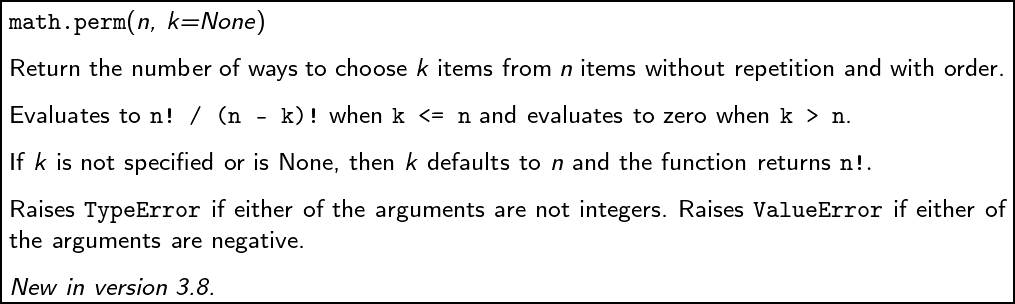In the documentation, we are told what the function does for each argument, and what exceptions may be raised.

## Summary

We have learned how to look up functions in the documentation for Python’s Standard Library, giving us access to a huge range of modules for everything from text processing, to graphics, to internet programming. In the next chapter, we will talk more about structuring the sort of larger programs we might write using a combination of our own functions and Standard Library functions.

The questions for this chapter use functions from the Standard Library, so you will need to have a copy of the documentation to hand.

## Questions

1. Compare the math.factorial function supplied with Python to the one we wrote in chapter 2. How do they differ?

2. Use the string module to write a function which detects if a given string represents a positive integer or not.

3. The function getpass.getpass from the getpass module can be used to accept input from the user without showing it on screen, in the manner in which we might type a password. Use this function to write a version of the guessing game from chapter 3 question 7 which allows one person to set up the guessing game in front of another, choosing the number to be guessed.

4. Use the statistics module to calculate the median, mode, and mean of a given list of numbers.

5. Use the functions time.time and time.sleep from the time module to write a reaction-time testing game.

# 12. Building Bigger Programs

We have been building progressively larger and larger programs, but they have all been run from Python’s interactive interpreter. Now, we shall write stand-alone programs, to be invoked at the command line. This means we can use them just like any other program on our computer, or share them with friends.

### Stand-alone programs

We wish to build stand-alone programs which we can run directly from the command line. The sys module provides the list sys.argv which contains, first, the name of the running script, and then any other arguments provided when the script was run. For example, consider the following program, saved as standalone.py: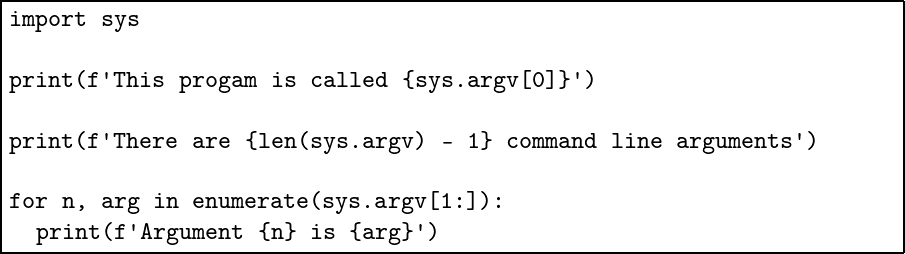We can run it and see what happens:

$python standalone.py This program is called standalone.py There are 0 command line arguments$ python standalone.py a b c
This program is called standalone.py
There are 3 command line arguments
Argument 0 is a
Argument 1 is b
Argument 2 is c

Remember that on some systems, you might need to type python3 instead of python. The $ is the command line prompt on the author’s computer – it may be different on yours. Now we can write stand-alone programs, to which we provide filenames and other arguments, instead of putting those details directly in the Python program itself. Much more flexible! ### A stand-alone text statistics program We shall now write a stand-alone version of out text statistics program from chapter 9. It will take the filename as an argument. In addition, we shall split our program into two: a file textstat.py to contain the bulk of the program, and another textstats.py to contain the part to do with command line arguments. Here is textstat.py: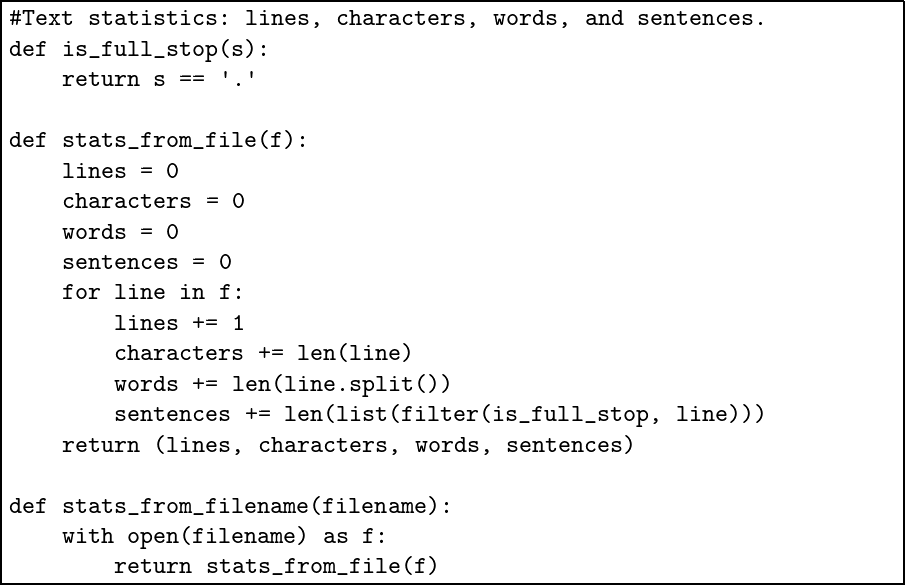Now, we can write the main program textstats.py, which will use the import keyword to access the stats_from_filename function of the textstat module.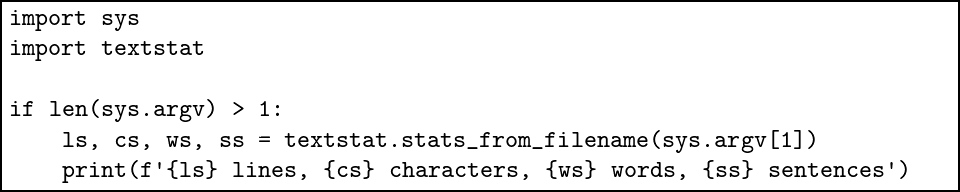The purpose of splitting the program this way is to allow the function stats_from_file and the function stats_from_filename to be used in other contexts without having to alter the whole program. Now we can run the program on its own, without loading an interactive Python session: $ python textstats.py gregor.txt
8 lines, 472 characters, 85 words, 4 sentences

In the questions, we will make stand-alone versions of some of our other programs, and some entirely new ones.

## Common problems

It is important, just as with any other list, to check that there is as much information as we expect in sys.argv, before looking up elements in it, or slicing it. If not, we can print out an error message, and a description of correct usage for the user. For example: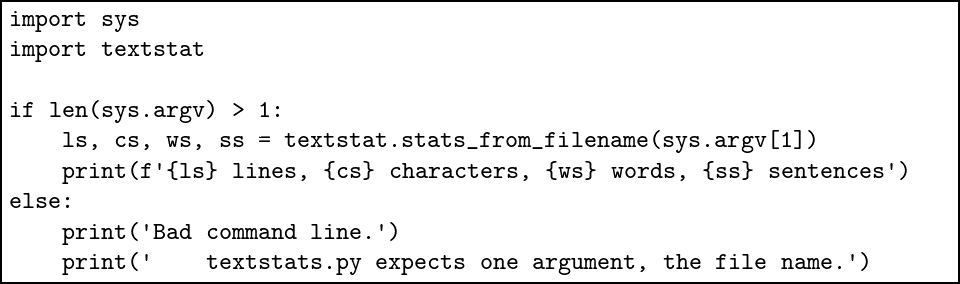## Summary

We have gone all the way from introducing addition in chapter 1, to building stand-alone programs in this chapter. We now have the tools to tackle larger projects, and that is what we shall be doing in the next four chapters.

## Questions

1. In question 3 of chapter 11 we updated our number-guessing game. Make a stand-alone program from this. It should take one argument, which is the maximum number. If no number is given, 100 is used as a default.

2. In question 5 of chapter 10 we wrote a function to plot a graph of a given function. Write a self-contained command line program to plot any function given as an argument, over a range similarly given. The built-in Python function eval can evaluate a given piece of Python program. For example, if the variable x has value 10 the result of eval(’x * 2’) is 20. Be sure to split your program into two modules: one to deal with the command line argument and one to do the graph plotting. Handle errors appropriately.

3. Write a simple note-taking program. When we run python note.py add todo "mow the lawn" the note mow the lawn should be added to the end of the file todo.txt. If the file does not exist, it should be created. Now extend the program to allow python note.py list which will list the notes by number. Running python note.py remove 4 should remove task number 4.

# Project 1: Pretty Pictures

So far we have been concerned only with programs which read and write text. But we have been sitting in front of a computer with graphical elements on the screen as well as textual ones.

There are many ways to produce pictures, both line drawing and photographic, using programming languages like Python. For this project, we will use the turtle module which uses a model of drawing invented for children but fun for adults too. In this model, there is a little ‘turtle‘ on screen, and we direct it where to go, and it leaves a trail behind it as it goes.

To begin, we import the turtle module, and create a new turtle, which we call t:

Python
>>> import turtle
>>> t = turtle.Turtle()

Upon typing the second line, a blank window appears, with the turtle represented by an arrow, pointing to the right: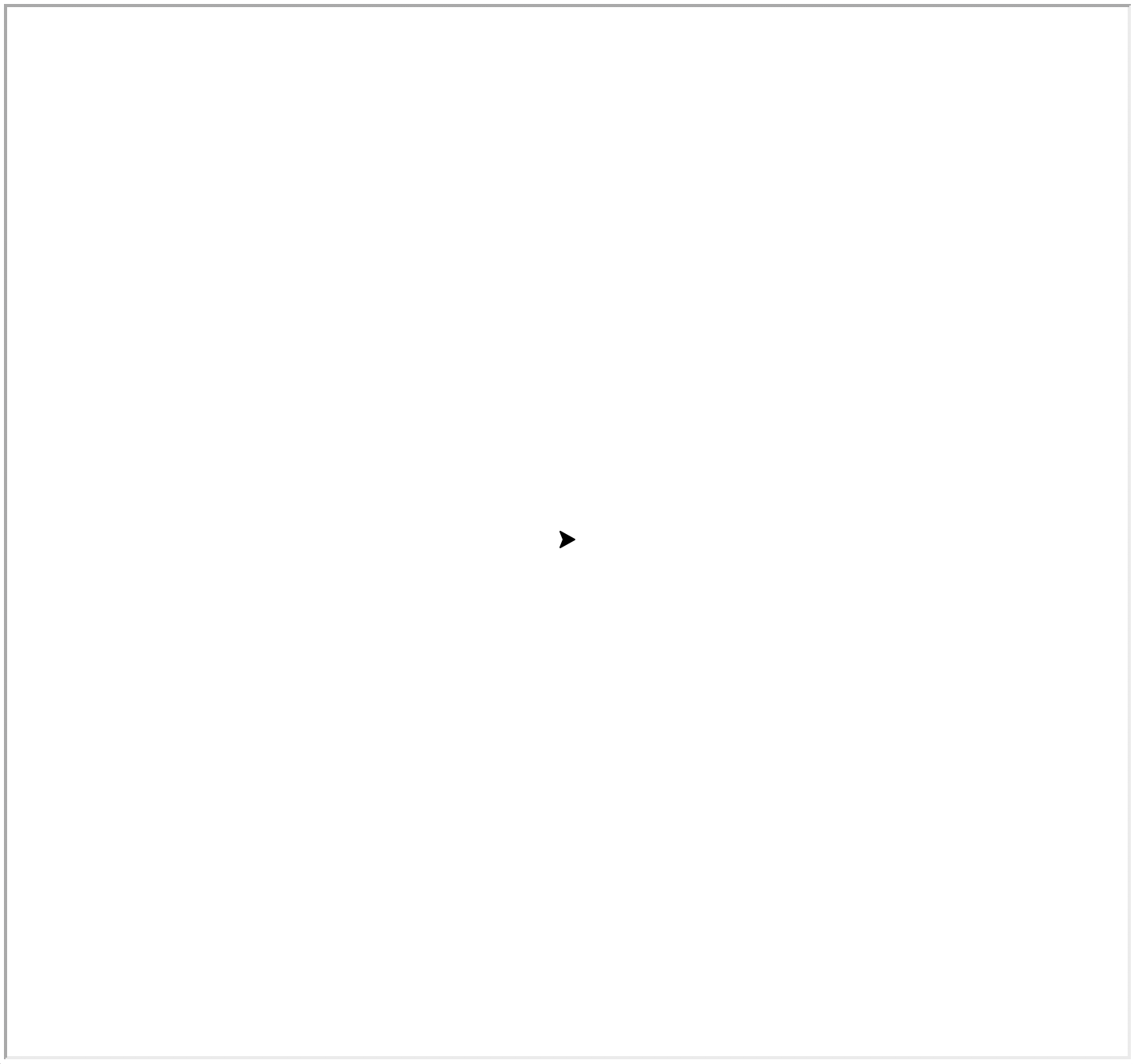We can now issue a command for the turtle to follow:

Python
>>> t.forward(100)

Here is the result: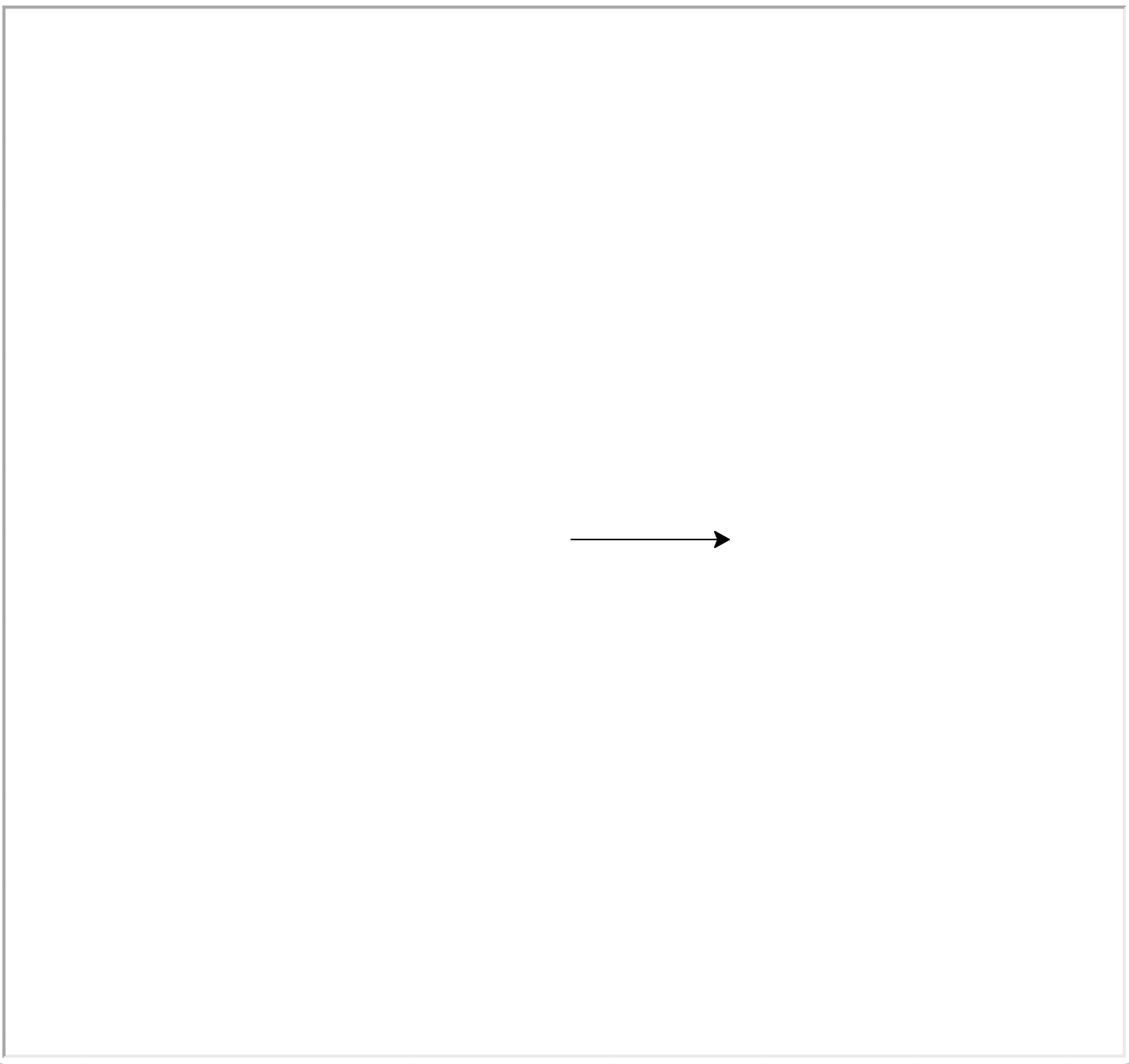We can complete the square by turning repeatedly by ninety degrees and moving forward.

Python
>>> t.right(90)
>>> t.forward(100)
>>> t.right(90)
>>> t.forward(100)
>>> t.right(90)
>>> t.forward(100)

The final result is a square of side 100, with the turtle in its original position, but pointing upwards: